Chapter 18 – Reinforcement Learning

This notebook contains all the sample code in chapter 18.

# Setup¶

First, let's import a few common modules, ensure MatplotLib plots figures inline and prepare a function to save the figures. We also check that Python 3.5 or later is installed (although Python 2.x may work, it is deprecated so we strongly recommend you use Python 3 instead), as well as Scikit-Learn ≥0.20 and TensorFlow ≥2.0.

In :
# Python ≥3.5 is required
import sys
assert sys.version_info >= (3, 5)

# Scikit-Learn ≥0.20 is required
import sklearn
assert sklearn.__version__ >= "0.20"

try:
# %tensorflow_version only exists in Colab.
%tensorflow_version 2.x
!apt update && apt install -y libpq-dev libsdl2-dev swig xorg-dev xvfb
!pip install -q -U tf-agents-nightly pyvirtualdisplay gym[atari]
IS_COLAB = True
except Exception:
IS_COLAB = False

# TensorFlow ≥2.0 is required
import tensorflow as tf
from tensorflow import keras
assert tf.__version__ >= "2.0"

if not tf.config.list_physical_devices('GPU'):
print("No GPU was detected. CNNs can be very slow without a GPU.")
if IS_COLAB:
print("Go to Runtime > Change runtime and select a GPU hardware accelerator.")

# Common imports
import numpy as np
import os

# to make this notebook's output stable across runs
np.random.seed(42)
tf.random.set_seed(42)

# To plot pretty figures
%matplotlib inline
import matplotlib as mpl
import matplotlib.pyplot as plt
mpl.rc('axes', labelsize=14)
mpl.rc('xtick', labelsize=12)
mpl.rc('ytick', labelsize=12)

# To get smooth animations
import matplotlib.animation as animation
mpl.rc('animation', html='jshtml')

# Where to save the figures
PROJECT_ROOT_DIR = "."
CHAPTER_ID = "rl"
IMAGES_PATH = os.path.join(PROJECT_ROOT_DIR, "images", CHAPTER_ID)
os.makedirs(IMAGES_PATH, exist_ok=True)

def save_fig(fig_id, tight_layout=True, fig_extension="png", resolution=300):
path = os.path.join(IMAGES_PATH, fig_id + "." + fig_extension)
print("Saving figure", fig_id)
if tight_layout:
plt.tight_layout()
plt.savefig(path, format=fig_extension, dpi=resolution)


# Introduction to OpenAI gym¶

In this notebook we will be using OpenAI gym, a great toolkit for developing and comparing Reinforcement Learning algorithms. It provides many environments for your learning agents to interact with. Let's start by importing gym:

In :
import gym


Let's list all the available environments:

In :
gym.envs.registry.all()

Out:
dict_values([EnvSpec(Copy-v0), EnvSpec(RepeatCopy-v0), EnvSpec(ReversedAddition-v0), EnvSpec(ReversedAddition3-v0), EnvSpec(DuplicatedInput-v0), EnvSpec(Reverse-v0), EnvSpec(CartPole-v0), EnvSpec(CartPole-v1), EnvSpec(MountainCar-v0), EnvSpec(MountainCarContinuous-v0), EnvSpec(Pendulum-v0), EnvSpec(Acrobot-v1), EnvSpec(LunarLander-v2), EnvSpec(LunarLanderContinuous-v2), EnvSpec(BipedalWalker-v3), EnvSpec(BipedalWalkerHardcore-v3), EnvSpec(CarRacing-v0), EnvSpec(Blackjack-v0), EnvSpec(KellyCoinflip-v0), EnvSpec(KellyCoinflipGeneralized-v0), EnvSpec(FrozenLake-v0), EnvSpec(FrozenLake8x8-v0), EnvSpec(CliffWalking-v0), EnvSpec(NChain-v0), EnvSpec(Roulette-v0), EnvSpec(Taxi-v3), EnvSpec(GuessingGame-v0), EnvSpec(HotterColder-v0), EnvSpec(Reacher-v2), EnvSpec(Pusher-v2), EnvSpec(Thrower-v2), EnvSpec(Striker-v2), EnvSpec(InvertedPendulum-v2), EnvSpec(InvertedDoublePendulum-v2), EnvSpec(HalfCheetah-v2), EnvSpec(HalfCheetah-v3), EnvSpec(Hopper-v2), EnvSpec(Hopper-v3), EnvSpec(Swimmer-v2), EnvSpec(Swimmer-v3), EnvSpec(Walker2d-v2), EnvSpec(Walker2d-v3), EnvSpec(Ant-v2), EnvSpec(Ant-v3), EnvSpec(Humanoid-v2), EnvSpec(Humanoid-v3), EnvSpec(HumanoidStandup-v2), EnvSpec(FetchSlide-v1), EnvSpec(FetchPickAndPlace-v1), EnvSpec(FetchReach-v1), EnvSpec(FetchPush-v1), EnvSpec(HandReach-v0), EnvSpec(HandManipulateBlockRotateZ-v0), EnvSpec(HandManipulateBlockRotateZTouchSensors-v0), EnvSpec(HandManipulateBlockRotateZTouchSensors-v1), EnvSpec(HandManipulateBlockRotateParallel-v0), EnvSpec(HandManipulateBlockRotateParallelTouchSensors-v0), EnvSpec(HandManipulateBlockRotateParallelTouchSensors-v1), EnvSpec(HandManipulateBlockRotateXYZ-v0), EnvSpec(HandManipulateBlockRotateXYZTouchSensors-v0), EnvSpec(HandManipulateBlockRotateXYZTouchSensors-v1), EnvSpec(HandManipulateBlockFull-v0), EnvSpec(HandManipulateBlock-v0), EnvSpec(HandManipulateBlockTouchSensors-v0), EnvSpec(HandManipulateBlockTouchSensors-v1), EnvSpec(HandManipulateEggRotate-v0), EnvSpec(HandManipulateEggRotateTouchSensors-v0), EnvSpec(HandManipulateEggRotateTouchSensors-v1), EnvSpec(HandManipulateEggFull-v0), EnvSpec(HandManipulateEgg-v0), EnvSpec(HandManipulateEggTouchSensors-v0), EnvSpec(HandManipulateEggTouchSensors-v1), EnvSpec(HandManipulatePenRotate-v0), EnvSpec(HandManipulatePenRotateTouchSensors-v0), EnvSpec(HandManipulatePenRotateTouchSensors-v1), EnvSpec(HandManipulatePenFull-v0), EnvSpec(HandManipulatePen-v0), EnvSpec(HandManipulatePenTouchSensors-v0), EnvSpec(HandManipulatePenTouchSensors-v1), EnvSpec(FetchSlideDense-v1), EnvSpec(FetchPickAndPlaceDense-v1), EnvSpec(FetchReachDense-v1), EnvSpec(FetchPushDense-v1), EnvSpec(HandReachDense-v0), EnvSpec(HandManipulateBlockRotateZDense-v0), EnvSpec(HandManipulateBlockRotateZTouchSensorsDense-v0), EnvSpec(HandManipulateBlockRotateZTouchSensorsDense-v1), EnvSpec(HandManipulateBlockRotateParallelDense-v0), EnvSpec(HandManipulateBlockRotateParallelTouchSensorsDense-v0), EnvSpec(HandManipulateBlockRotateParallelTouchSensorsDense-v1), EnvSpec(HandManipulateBlockRotateXYZDense-v0), EnvSpec(HandManipulateBlockRotateXYZTouchSensorsDense-v0), EnvSpec(HandManipulateBlockRotateXYZTouchSensorsDense-v1), EnvSpec(HandManipulateBlockFullDense-v0), EnvSpec(HandManipulateBlockDense-v0), EnvSpec(HandManipulateBlockTouchSensorsDense-v0), EnvSpec(HandManipulateBlockTouchSensorsDense-v1), EnvSpec(HandManipulateEggRotateDense-v0), EnvSpec(HandManipulateEggRotateTouchSensorsDense-v0), EnvSpec(HandManipulateEggRotateTouchSensorsDense-v1), EnvSpec(HandManipulateEggFullDense-v0), EnvSpec(HandManipulateEggDense-v0), EnvSpec(HandManipulateEggTouchSensorsDense-v0), EnvSpec(HandManipulateEggTouchSensorsDense-v1), EnvSpec(HandManipulatePenRotateDense-v0), EnvSpec(HandManipulatePenRotateTouchSensorsDense-v0), EnvSpec(HandManipulatePenRotateTouchSensorsDense-v1), EnvSpec(HandManipulatePenFullDense-v0), EnvSpec(HandManipulatePenDense-v0), EnvSpec(HandManipulatePenTouchSensorsDense-v0), EnvSpec(HandManipulatePenTouchSensorsDense-v1), EnvSpec(Adventure-v0), EnvSpec(Adventure-v4), EnvSpec(AdventureDeterministic-v0), EnvSpec(AdventureDeterministic-v4), EnvSpec(AdventureNoFrameskip-v0), EnvSpec(AdventureNoFrameskip-v4), EnvSpec(Adventure-ram-v0), EnvSpec(Adventure-ram-v4), EnvSpec(Adventure-ramDeterministic-v0), EnvSpec(Adventure-ramDeterministic-v4), EnvSpec(Adventure-ramNoFrameskip-v0), EnvSpec(Adventure-ramNoFrameskip-v4), EnvSpec(AirRaid-v0), EnvSpec(AirRaid-v4), EnvSpec(AirRaidDeterministic-v0), EnvSpec(AirRaidDeterministic-v4), EnvSpec(AirRaidNoFrameskip-v0), EnvSpec(AirRaidNoFrameskip-v4), EnvSpec(AirRaid-ram-v0), EnvSpec(AirRaid-ram-v4), EnvSpec(AirRaid-ramDeterministic-v0), EnvSpec(AirRaid-ramDeterministic-v4), EnvSpec(AirRaid-ramNoFrameskip-v0), EnvSpec(AirRaid-ramNoFrameskip-v4), EnvSpec(Alien-v0), EnvSpec(Alien-v4), EnvSpec(AlienDeterministic-v0), EnvSpec(AlienDeterministic-v4), EnvSpec(AlienNoFrameskip-v0), EnvSpec(AlienNoFrameskip-v4), EnvSpec(Alien-ram-v0), EnvSpec(Alien-ram-v4), EnvSpec(Alien-ramDeterministic-v0), EnvSpec(Alien-ramDeterministic-v4), EnvSpec(Alien-ramNoFrameskip-v0), EnvSpec(Alien-ramNoFrameskip-v4), EnvSpec(Amidar-v0), EnvSpec(Amidar-v4), EnvSpec(AmidarDeterministic-v0), EnvSpec(AmidarDeterministic-v4), EnvSpec(AmidarNoFrameskip-v0), EnvSpec(AmidarNoFrameskip-v4), EnvSpec(Amidar-ram-v0), EnvSpec(Amidar-ram-v4), EnvSpec(Amidar-ramDeterministic-v0), EnvSpec(Amidar-ramDeterministic-v4), EnvSpec(Amidar-ramNoFrameskip-v0), EnvSpec(Amidar-ramNoFrameskip-v4), EnvSpec(Assault-v0), EnvSpec(Assault-v4), EnvSpec(AssaultDeterministic-v0), EnvSpec(AssaultDeterministic-v4), EnvSpec(AssaultNoFrameskip-v0), EnvSpec(AssaultNoFrameskip-v4), EnvSpec(Assault-ram-v0), EnvSpec(Assault-ram-v4), EnvSpec(Assault-ramDeterministic-v0), EnvSpec(Assault-ramDeterministic-v4), EnvSpec(Assault-ramNoFrameskip-v0), EnvSpec(Assault-ramNoFrameskip-v4), EnvSpec(Asterix-v0), EnvSpec(Asterix-v4), EnvSpec(AsterixDeterministic-v0), EnvSpec(AsterixDeterministic-v4), EnvSpec(AsterixNoFrameskip-v0), EnvSpec(AsterixNoFrameskip-v4), EnvSpec(Asterix-ram-v0), EnvSpec(Asterix-ram-v4), EnvSpec(Asterix-ramDeterministic-v0), EnvSpec(Asterix-ramDeterministic-v4), EnvSpec(Asterix-ramNoFrameskip-v0), EnvSpec(Asterix-ramNoFrameskip-v4), EnvSpec(Asteroids-v0), EnvSpec(Asteroids-v4), EnvSpec(AsteroidsDeterministic-v0), EnvSpec(AsteroidsDeterministic-v4), EnvSpec(AsteroidsNoFrameskip-v0), EnvSpec(AsteroidsNoFrameskip-v4), EnvSpec(Asteroids-ram-v0), EnvSpec(Asteroids-ram-v4), EnvSpec(Asteroids-ramDeterministic-v0), EnvSpec(Asteroids-ramDeterministic-v4), EnvSpec(Asteroids-ramNoFrameskip-v0), EnvSpec(Asteroids-ramNoFrameskip-v4), EnvSpec(Atlantis-v0), EnvSpec(Atlantis-v4), EnvSpec(AtlantisDeterministic-v0), EnvSpec(AtlantisDeterministic-v4), EnvSpec(AtlantisNoFrameskip-v0), EnvSpec(AtlantisNoFrameskip-v4), EnvSpec(Atlantis-ram-v0), EnvSpec(Atlantis-ram-v4), EnvSpec(Atlantis-ramDeterministic-v0), EnvSpec(Atlantis-ramDeterministic-v4), EnvSpec(Atlantis-ramNoFrameskip-v0), EnvSpec(Atlantis-ramNoFrameskip-v4), EnvSpec(BankHeist-v0), EnvSpec(BankHeist-v4), EnvSpec(BankHeistDeterministic-v0), EnvSpec(BankHeistDeterministic-v4), EnvSpec(BankHeistNoFrameskip-v0), EnvSpec(BankHeistNoFrameskip-v4), EnvSpec(BankHeist-ram-v0), EnvSpec(BankHeist-ram-v4), EnvSpec(BankHeist-ramDeterministic-v0), EnvSpec(BankHeist-ramDeterministic-v4), EnvSpec(BankHeist-ramNoFrameskip-v0), EnvSpec(BankHeist-ramNoFrameskip-v4), EnvSpec(BattleZone-v0), EnvSpec(BattleZone-v4), EnvSpec(BattleZoneDeterministic-v0), EnvSpec(BattleZoneDeterministic-v4), EnvSpec(BattleZoneNoFrameskip-v0), EnvSpec(BattleZoneNoFrameskip-v4), EnvSpec(BattleZone-ram-v0), EnvSpec(BattleZone-ram-v4), EnvSpec(BattleZone-ramDeterministic-v0), EnvSpec(BattleZone-ramDeterministic-v4), EnvSpec(BattleZone-ramNoFrameskip-v0), EnvSpec(BattleZone-ramNoFrameskip-v4), EnvSpec(BeamRider-v0), EnvSpec(BeamRider-v4), EnvSpec(BeamRiderDeterministic-v0), EnvSpec(BeamRiderDeterministic-v4), EnvSpec(BeamRiderNoFrameskip-v0), EnvSpec(BeamRiderNoFrameskip-v4), EnvSpec(BeamRider-ram-v0), EnvSpec(BeamRider-ram-v4), EnvSpec(BeamRider-ramDeterministic-v0), EnvSpec(BeamRider-ramDeterministic-v4), EnvSpec(BeamRider-ramNoFrameskip-v0), EnvSpec(BeamRider-ramNoFrameskip-v4), EnvSpec(Berzerk-v0), EnvSpec(Berzerk-v4), EnvSpec(BerzerkDeterministic-v0), EnvSpec(BerzerkDeterministic-v4), EnvSpec(BerzerkNoFrameskip-v0), EnvSpec(BerzerkNoFrameskip-v4), EnvSpec(Berzerk-ram-v0), EnvSpec(Berzerk-ram-v4), EnvSpec(Berzerk-ramDeterministic-v0), EnvSpec(Berzerk-ramDeterministic-v4), EnvSpec(Berzerk-ramNoFrameskip-v0), EnvSpec(Berzerk-ramNoFrameskip-v4), EnvSpec(Bowling-v0), EnvSpec(Bowling-v4), EnvSpec(BowlingDeterministic-v0), EnvSpec(BowlingDeterministic-v4), EnvSpec(BowlingNoFrameskip-v0), EnvSpec(BowlingNoFrameskip-v4), EnvSpec(Bowling-ram-v0), EnvSpec(Bowling-ram-v4), EnvSpec(Bowling-ramDeterministic-v0), EnvSpec(Bowling-ramDeterministic-v4), EnvSpec(Bowling-ramNoFrameskip-v0), EnvSpec(Bowling-ramNoFrameskip-v4), EnvSpec(Boxing-v0), EnvSpec(Boxing-v4), EnvSpec(BoxingDeterministic-v0), EnvSpec(BoxingDeterministic-v4), EnvSpec(BoxingNoFrameskip-v0), EnvSpec(BoxingNoFrameskip-v4), EnvSpec(Boxing-ram-v0), EnvSpec(Boxing-ram-v4), EnvSpec(Boxing-ramDeterministic-v0), EnvSpec(Boxing-ramDeterministic-v4), EnvSpec(Boxing-ramNoFrameskip-v0), EnvSpec(Boxing-ramNoFrameskip-v4), EnvSpec(Breakout-v0), EnvSpec(Breakout-v4), EnvSpec(BreakoutDeterministic-v0), EnvSpec(BreakoutDeterministic-v4), EnvSpec(BreakoutNoFrameskip-v0), EnvSpec(BreakoutNoFrameskip-v4), EnvSpec(Breakout-ram-v0), EnvSpec(Breakout-ram-v4), EnvSpec(Breakout-ramDeterministic-v0), EnvSpec(Breakout-ramDeterministic-v4), EnvSpec(Breakout-ramNoFrameskip-v0), EnvSpec(Breakout-ramNoFrameskip-v4), EnvSpec(Carnival-v0), EnvSpec(Carnival-v4), EnvSpec(CarnivalDeterministic-v0), EnvSpec(CarnivalDeterministic-v4), EnvSpec(CarnivalNoFrameskip-v0), EnvSpec(CarnivalNoFrameskip-v4), EnvSpec(Carnival-ram-v0), EnvSpec(Carnival-ram-v4), EnvSpec(Carnival-ramDeterministic-v0), EnvSpec(Carnival-ramDeterministic-v4), EnvSpec(Carnival-ramNoFrameskip-v0), EnvSpec(Carnival-ramNoFrameskip-v4), EnvSpec(Centipede-v0), EnvSpec(Centipede-v4), EnvSpec(CentipedeDeterministic-v0), EnvSpec(CentipedeDeterministic-v4), EnvSpec(CentipedeNoFrameskip-v0), EnvSpec(CentipedeNoFrameskip-v4), EnvSpec(Centipede-ram-v0), EnvSpec(Centipede-ram-v4), EnvSpec(Centipede-ramDeterministic-v0), EnvSpec(Centipede-ramDeterministic-v4), EnvSpec(Centipede-ramNoFrameskip-v0), EnvSpec(Centipede-ramNoFrameskip-v4), EnvSpec(ChopperCommand-v0), EnvSpec(ChopperCommand-v4), EnvSpec(ChopperCommandDeterministic-v0), EnvSpec(ChopperCommandDeterministic-v4), EnvSpec(ChopperCommandNoFrameskip-v0), EnvSpec(ChopperCommandNoFrameskip-v4), EnvSpec(ChopperCommand-ram-v0), EnvSpec(ChopperCommand-ram-v4), EnvSpec(ChopperCommand-ramDeterministic-v0), EnvSpec(ChopperCommand-ramDeterministic-v4), EnvSpec(ChopperCommand-ramNoFrameskip-v0), EnvSpec(ChopperCommand-ramNoFrameskip-v4), EnvSpec(CrazyClimber-v0), EnvSpec(CrazyClimber-v4), EnvSpec(CrazyClimberDeterministic-v0), EnvSpec(CrazyClimberDeterministic-v4), EnvSpec(CrazyClimberNoFrameskip-v0), EnvSpec(CrazyClimberNoFrameskip-v4), EnvSpec(CrazyClimber-ram-v0), EnvSpec(CrazyClimber-ram-v4), EnvSpec(CrazyClimber-ramDeterministic-v0), EnvSpec(CrazyClimber-ramDeterministic-v4), EnvSpec(CrazyClimber-ramNoFrameskip-v0), EnvSpec(CrazyClimber-ramNoFrameskip-v4), EnvSpec(Defender-v0), EnvSpec(Defender-v4), EnvSpec(DefenderDeterministic-v0), EnvSpec(DefenderDeterministic-v4), EnvSpec(DefenderNoFrameskip-v0), EnvSpec(DefenderNoFrameskip-v4), EnvSpec(Defender-ram-v0), EnvSpec(Defender-ram-v4), EnvSpec(Defender-ramDeterministic-v0), EnvSpec(Defender-ramDeterministic-v4), EnvSpec(Defender-ramNoFrameskip-v0), EnvSpec(Defender-ramNoFrameskip-v4), EnvSpec(DemonAttack-v0), EnvSpec(DemonAttack-v4), EnvSpec(DemonAttackDeterministic-v0), EnvSpec(DemonAttackDeterministic-v4), EnvSpec(DemonAttackNoFrameskip-v0), EnvSpec(DemonAttackNoFrameskip-v4), EnvSpec(DemonAttack-ram-v0), EnvSpec(DemonAttack-ram-v4), EnvSpec(DemonAttack-ramDeterministic-v0), EnvSpec(DemonAttack-ramDeterministic-v4), EnvSpec(DemonAttack-ramNoFrameskip-v0), EnvSpec(DemonAttack-ramNoFrameskip-v4), EnvSpec(DoubleDunk-v0), EnvSpec(DoubleDunk-v4), EnvSpec(DoubleDunkDeterministic-v0), EnvSpec(DoubleDunkDeterministic-v4), EnvSpec(DoubleDunkNoFrameskip-v0), EnvSpec(DoubleDunkNoFrameskip-v4), EnvSpec(DoubleDunk-ram-v0), EnvSpec(DoubleDunk-ram-v4), EnvSpec(DoubleDunk-ramDeterministic-v0), EnvSpec(DoubleDunk-ramDeterministic-v4), EnvSpec(DoubleDunk-ramNoFrameskip-v0), EnvSpec(DoubleDunk-ramNoFrameskip-v4), EnvSpec(ElevatorAction-v0), EnvSpec(ElevatorAction-v4), EnvSpec(ElevatorActionDeterministic-v0), EnvSpec(ElevatorActionDeterministic-v4), EnvSpec(ElevatorActionNoFrameskip-v0), EnvSpec(ElevatorActionNoFrameskip-v4), EnvSpec(ElevatorAction-ram-v0), EnvSpec(ElevatorAction-ram-v4), EnvSpec(ElevatorAction-ramDeterministic-v0), EnvSpec(ElevatorAction-ramDeterministic-v4), EnvSpec(ElevatorAction-ramNoFrameskip-v0), EnvSpec(ElevatorAction-ramNoFrameskip-v4), EnvSpec(Enduro-v0), EnvSpec(Enduro-v4), EnvSpec(EnduroDeterministic-v0), EnvSpec(EnduroDeterministic-v4), EnvSpec(EnduroNoFrameskip-v0), EnvSpec(EnduroNoFrameskip-v4), EnvSpec(Enduro-ram-v0), EnvSpec(Enduro-ram-v4), EnvSpec(Enduro-ramDeterministic-v0), EnvSpec(Enduro-ramDeterministic-v4), EnvSpec(Enduro-ramNoFrameskip-v0), EnvSpec(Enduro-ramNoFrameskip-v4), EnvSpec(FishingDerby-v0), EnvSpec(FishingDerby-v4), EnvSpec(FishingDerbyDeterministic-v0), EnvSpec(FishingDerbyDeterministic-v4), EnvSpec(FishingDerbyNoFrameskip-v0), EnvSpec(FishingDerbyNoFrameskip-v4), EnvSpec(FishingDerby-ram-v0), EnvSpec(FishingDerby-ram-v4), EnvSpec(FishingDerby-ramDeterministic-v0), EnvSpec(FishingDerby-ramDeterministic-v4), EnvSpec(FishingDerby-ramNoFrameskip-v0), EnvSpec(FishingDerby-ramNoFrameskip-v4), EnvSpec(Freeway-v0), EnvSpec(Freeway-v4), EnvSpec(FreewayDeterministic-v0), EnvSpec(FreewayDeterministic-v4), EnvSpec(FreewayNoFrameskip-v0), EnvSpec(FreewayNoFrameskip-v4), EnvSpec(Freeway-ram-v0), EnvSpec(Freeway-ram-v4), EnvSpec(Freeway-ramDeterministic-v0), EnvSpec(Freeway-ramDeterministic-v4), EnvSpec(Freeway-ramNoFrameskip-v0), EnvSpec(Freeway-ramNoFrameskip-v4), EnvSpec(Frostbite-v0), EnvSpec(Frostbite-v4), EnvSpec(FrostbiteDeterministic-v0), EnvSpec(FrostbiteDeterministic-v4), EnvSpec(FrostbiteNoFrameskip-v0), EnvSpec(FrostbiteNoFrameskip-v4), EnvSpec(Frostbite-ram-v0), EnvSpec(Frostbite-ram-v4), EnvSpec(Frostbite-ramDeterministic-v0), EnvSpec(Frostbite-ramDeterministic-v4), EnvSpec(Frostbite-ramNoFrameskip-v0), EnvSpec(Frostbite-ramNoFrameskip-v4), EnvSpec(Gopher-v0), EnvSpec(Gopher-v4), EnvSpec(GopherDeterministic-v0), EnvSpec(GopherDeterministic-v4), EnvSpec(GopherNoFrameskip-v0), EnvSpec(GopherNoFrameskip-v4), EnvSpec(Gopher-ram-v0), EnvSpec(Gopher-ram-v4), EnvSpec(Gopher-ramDeterministic-v0), EnvSpec(Gopher-ramDeterministic-v4), EnvSpec(Gopher-ramNoFrameskip-v0), EnvSpec(Gopher-ramNoFrameskip-v4), EnvSpec(Gravitar-v0), EnvSpec(Gravitar-v4), EnvSpec(GravitarDeterministic-v0), EnvSpec(GravitarDeterministic-v4), EnvSpec(GravitarNoFrameskip-v0), EnvSpec(GravitarNoFrameskip-v4), EnvSpec(Gravitar-ram-v0), EnvSpec(Gravitar-ram-v4), EnvSpec(Gravitar-ramDeterministic-v0), EnvSpec(Gravitar-ramDeterministic-v4), EnvSpec(Gravitar-ramNoFrameskip-v0), EnvSpec(Gravitar-ramNoFrameskip-v4), EnvSpec(Hero-v0), EnvSpec(Hero-v4), EnvSpec(HeroDeterministic-v0), EnvSpec(HeroDeterministic-v4), EnvSpec(HeroNoFrameskip-v0), EnvSpec(HeroNoFrameskip-v4), EnvSpec(Hero-ram-v0), EnvSpec(Hero-ram-v4), EnvSpec(Hero-ramDeterministic-v0), EnvSpec(Hero-ramDeterministic-v4), EnvSpec(Hero-ramNoFrameskip-v0), EnvSpec(Hero-ramNoFrameskip-v4), EnvSpec(IceHockey-v0), EnvSpec(IceHockey-v4), EnvSpec(IceHockeyDeterministic-v0), EnvSpec(IceHockeyDeterministic-v4), EnvSpec(IceHockeyNoFrameskip-v0), EnvSpec(IceHockeyNoFrameskip-v4), EnvSpec(IceHockey-ram-v0), EnvSpec(IceHockey-ram-v4), EnvSpec(IceHockey-ramDeterministic-v0), EnvSpec(IceHockey-ramDeterministic-v4), EnvSpec(IceHockey-ramNoFrameskip-v0), EnvSpec(IceHockey-ramNoFrameskip-v4), EnvSpec(Jamesbond-v0), EnvSpec(Jamesbond-v4), EnvSpec(JamesbondDeterministic-v0), EnvSpec(JamesbondDeterministic-v4), EnvSpec(JamesbondNoFrameskip-v0), EnvSpec(JamesbondNoFrameskip-v4), EnvSpec(Jamesbond-ram-v0), EnvSpec(Jamesbond-ram-v4), EnvSpec(Jamesbond-ramDeterministic-v0), EnvSpec(Jamesbond-ramDeterministic-v4), EnvSpec(Jamesbond-ramNoFrameskip-v0), EnvSpec(Jamesbond-ramNoFrameskip-v4), EnvSpec(JourneyEscape-v0), EnvSpec(JourneyEscape-v4), EnvSpec(JourneyEscapeDeterministic-v0), EnvSpec(JourneyEscapeDeterministic-v4), EnvSpec(JourneyEscapeNoFrameskip-v0), EnvSpec(JourneyEscapeNoFrameskip-v4), EnvSpec(JourneyEscape-ram-v0), EnvSpec(JourneyEscape-ram-v4), EnvSpec(JourneyEscape-ramDeterministic-v0), EnvSpec(JourneyEscape-ramDeterministic-v4), EnvSpec(JourneyEscape-ramNoFrameskip-v0), EnvSpec(JourneyEscape-ramNoFrameskip-v4), EnvSpec(Kangaroo-v0), EnvSpec(Kangaroo-v4), EnvSpec(KangarooDeterministic-v0), EnvSpec(KangarooDeterministic-v4), EnvSpec(KangarooNoFrameskip-v0), EnvSpec(KangarooNoFrameskip-v4), EnvSpec(Kangaroo-ram-v0), EnvSpec(Kangaroo-ram-v4), EnvSpec(Kangaroo-ramDeterministic-v0), EnvSpec(Kangaroo-ramDeterministic-v4), EnvSpec(Kangaroo-ramNoFrameskip-v0), EnvSpec(Kangaroo-ramNoFrameskip-v4), EnvSpec(Krull-v0), EnvSpec(Krull-v4), EnvSpec(KrullDeterministic-v0), EnvSpec(KrullDeterministic-v4), EnvSpec(KrullNoFrameskip-v0), EnvSpec(KrullNoFrameskip-v4), EnvSpec(Krull-ram-v0), EnvSpec(Krull-ram-v4), EnvSpec(Krull-ramDeterministic-v0), EnvSpec(Krull-ramDeterministic-v4), EnvSpec(Krull-ramNoFrameskip-v0), EnvSpec(Krull-ramNoFrameskip-v4), EnvSpec(KungFuMaster-v0), EnvSpec(KungFuMaster-v4), EnvSpec(KungFuMasterDeterministic-v0), EnvSpec(KungFuMasterDeterministic-v4), EnvSpec(KungFuMasterNoFrameskip-v0), EnvSpec(KungFuMasterNoFrameskip-v4), EnvSpec(KungFuMaster-ram-v0), EnvSpec(KungFuMaster-ram-v4), EnvSpec(KungFuMaster-ramDeterministic-v0), EnvSpec(KungFuMaster-ramDeterministic-v4), EnvSpec(KungFuMaster-ramNoFrameskip-v0), EnvSpec(KungFuMaster-ramNoFrameskip-v4), EnvSpec(MontezumaRevenge-v0), EnvSpec(MontezumaRevenge-v4), EnvSpec(MontezumaRevengeDeterministic-v0), EnvSpec(MontezumaRevengeDeterministic-v4), EnvSpec(MontezumaRevengeNoFrameskip-v0), EnvSpec(MontezumaRevengeNoFrameskip-v4), EnvSpec(MontezumaRevenge-ram-v0), EnvSpec(MontezumaRevenge-ram-v4), EnvSpec(MontezumaRevenge-ramDeterministic-v0), EnvSpec(MontezumaRevenge-ramDeterministic-v4), EnvSpec(MontezumaRevenge-ramNoFrameskip-v0), EnvSpec(MontezumaRevenge-ramNoFrameskip-v4), EnvSpec(MsPacman-v0), EnvSpec(MsPacman-v4), EnvSpec(MsPacmanDeterministic-v0), EnvSpec(MsPacmanDeterministic-v4), EnvSpec(MsPacmanNoFrameskip-v0), EnvSpec(MsPacmanNoFrameskip-v4), EnvSpec(MsPacman-ram-v0), EnvSpec(MsPacman-ram-v4), EnvSpec(MsPacman-ramDeterministic-v0), EnvSpec(MsPacman-ramDeterministic-v4), EnvSpec(MsPacman-ramNoFrameskip-v0), EnvSpec(MsPacman-ramNoFrameskip-v4), EnvSpec(NameThisGame-v0), EnvSpec(NameThisGame-v4), EnvSpec(NameThisGameDeterministic-v0), EnvSpec(NameThisGameDeterministic-v4), EnvSpec(NameThisGameNoFrameskip-v0), EnvSpec(NameThisGameNoFrameskip-v4), EnvSpec(NameThisGame-ram-v0), EnvSpec(NameThisGame-ram-v4), EnvSpec(NameThisGame-ramDeterministic-v0), EnvSpec(NameThisGame-ramDeterministic-v4), EnvSpec(NameThisGame-ramNoFrameskip-v0), EnvSpec(NameThisGame-ramNoFrameskip-v4), EnvSpec(Phoenix-v0), EnvSpec(Phoenix-v4), EnvSpec(PhoenixDeterministic-v0), EnvSpec(PhoenixDeterministic-v4), EnvSpec(PhoenixNoFrameskip-v0), EnvSpec(PhoenixNoFrameskip-v4), EnvSpec(Phoenix-ram-v0), EnvSpec(Phoenix-ram-v4), EnvSpec(Phoenix-ramDeterministic-v0), EnvSpec(Phoenix-ramDeterministic-v4), EnvSpec(Phoenix-ramNoFrameskip-v0), EnvSpec(Phoenix-ramNoFrameskip-v4), EnvSpec(Pitfall-v0), EnvSpec(Pitfall-v4), EnvSpec(PitfallDeterministic-v0), EnvSpec(PitfallDeterministic-v4), EnvSpec(PitfallNoFrameskip-v0), EnvSpec(PitfallNoFrameskip-v4), EnvSpec(Pitfall-ram-v0), EnvSpec(Pitfall-ram-v4), EnvSpec(Pitfall-ramDeterministic-v0), EnvSpec(Pitfall-ramDeterministic-v4), EnvSpec(Pitfall-ramNoFrameskip-v0), EnvSpec(Pitfall-ramNoFrameskip-v4), EnvSpec(Pong-v0), EnvSpec(Pong-v4), EnvSpec(PongDeterministic-v0), EnvSpec(PongDeterministic-v4), EnvSpec(PongNoFrameskip-v0), EnvSpec(PongNoFrameskip-v4), EnvSpec(Pong-ram-v0), EnvSpec(Pong-ram-v4), EnvSpec(Pong-ramDeterministic-v0), EnvSpec(Pong-ramDeterministic-v4), EnvSpec(Pong-ramNoFrameskip-v0), EnvSpec(Pong-ramNoFrameskip-v4), EnvSpec(Pooyan-v0), EnvSpec(Pooyan-v4), EnvSpec(PooyanDeterministic-v0), EnvSpec(PooyanDeterministic-v4), EnvSpec(PooyanNoFrameskip-v0), EnvSpec(PooyanNoFrameskip-v4), EnvSpec(Pooyan-ram-v0), EnvSpec(Pooyan-ram-v4), EnvSpec(Pooyan-ramDeterministic-v0), EnvSpec(Pooyan-ramDeterministic-v4), EnvSpec(Pooyan-ramNoFrameskip-v0), EnvSpec(Pooyan-ramNoFrameskip-v4), EnvSpec(PrivateEye-v0), EnvSpec(PrivateEye-v4), EnvSpec(PrivateEyeDeterministic-v0), EnvSpec(PrivateEyeDeterministic-v4), EnvSpec(PrivateEyeNoFrameskip-v0), EnvSpec(PrivateEyeNoFrameskip-v4), EnvSpec(PrivateEye-ram-v0), EnvSpec(PrivateEye-ram-v4), EnvSpec(PrivateEye-ramDeterministic-v0), EnvSpec(PrivateEye-ramDeterministic-v4), EnvSpec(PrivateEye-ramNoFrameskip-v0), EnvSpec(PrivateEye-ramNoFrameskip-v4), EnvSpec(Qbert-v0), EnvSpec(Qbert-v4), EnvSpec(QbertDeterministic-v0), EnvSpec(QbertDeterministic-v4), EnvSpec(QbertNoFrameskip-v0), EnvSpec(QbertNoFrameskip-v4), EnvSpec(Qbert-ram-v0), EnvSpec(Qbert-ram-v4), EnvSpec(Qbert-ramDeterministic-v0), EnvSpec(Qbert-ramDeterministic-v4), EnvSpec(Qbert-ramNoFrameskip-v0), EnvSpec(Qbert-ramNoFrameskip-v4), EnvSpec(Riverraid-v0), EnvSpec(Riverraid-v4), EnvSpec(RiverraidDeterministic-v0), EnvSpec(RiverraidDeterministic-v4), EnvSpec(RiverraidNoFrameskip-v0), EnvSpec(RiverraidNoFrameskip-v4), EnvSpec(Riverraid-ram-v0), EnvSpec(Riverraid-ram-v4), EnvSpec(Riverraid-ramDeterministic-v0), EnvSpec(Riverraid-ramDeterministic-v4), EnvSpec(Riverraid-ramNoFrameskip-v0), EnvSpec(Riverraid-ramNoFrameskip-v4), EnvSpec(RoadRunner-v0), EnvSpec(RoadRunner-v4), EnvSpec(RoadRunnerDeterministic-v0), EnvSpec(RoadRunnerDeterministic-v4), EnvSpec(RoadRunnerNoFrameskip-v0), EnvSpec(RoadRunnerNoFrameskip-v4), EnvSpec(RoadRunner-ram-v0), EnvSpec(RoadRunner-ram-v4), EnvSpec(RoadRunner-ramDeterministic-v0), EnvSpec(RoadRunner-ramDeterministic-v4), EnvSpec(RoadRunner-ramNoFrameskip-v0), EnvSpec(RoadRunner-ramNoFrameskip-v4), EnvSpec(Robotank-v0), EnvSpec(Robotank-v4), EnvSpec(RobotankDeterministic-v0), EnvSpec(RobotankDeterministic-v4), EnvSpec(RobotankNoFrameskip-v0), EnvSpec(RobotankNoFrameskip-v4), EnvSpec(Robotank-ram-v0), EnvSpec(Robotank-ram-v4), EnvSpec(Robotank-ramDeterministic-v0), EnvSpec(Robotank-ramDeterministic-v4), EnvSpec(Robotank-ramNoFrameskip-v0), EnvSpec(Robotank-ramNoFrameskip-v4), EnvSpec(Seaquest-v0), EnvSpec(Seaquest-v4), EnvSpec(SeaquestDeterministic-v0), EnvSpec(SeaquestDeterministic-v4), EnvSpec(SeaquestNoFrameskip-v0), EnvSpec(SeaquestNoFrameskip-v4), EnvSpec(Seaquest-ram-v0), EnvSpec(Seaquest-ram-v4), EnvSpec(Seaquest-ramDeterministic-v0), EnvSpec(Seaquest-ramDeterministic-v4), EnvSpec(Seaquest-ramNoFrameskip-v0), EnvSpec(Seaquest-ramNoFrameskip-v4), EnvSpec(Skiing-v0), EnvSpec(Skiing-v4), EnvSpec(SkiingDeterministic-v0), EnvSpec(SkiingDeterministic-v4), EnvSpec(SkiingNoFrameskip-v0), EnvSpec(SkiingNoFrameskip-v4), EnvSpec(Skiing-ram-v0), EnvSpec(Skiing-ram-v4), EnvSpec(Skiing-ramDeterministic-v0), EnvSpec(Skiing-ramDeterministic-v4), EnvSpec(Skiing-ramNoFrameskip-v0), EnvSpec(Skiing-ramNoFrameskip-v4), EnvSpec(Solaris-v0), EnvSpec(Solaris-v4), EnvSpec(SolarisDeterministic-v0), EnvSpec(SolarisDeterministic-v4), EnvSpec(SolarisNoFrameskip-v0), EnvSpec(SolarisNoFrameskip-v4), EnvSpec(Solaris-ram-v0), EnvSpec(Solaris-ram-v4), EnvSpec(Solaris-ramDeterministic-v0), EnvSpec(Solaris-ramDeterministic-v4), EnvSpec(Solaris-ramNoFrameskip-v0), EnvSpec(Solaris-ramNoFrameskip-v4), EnvSpec(SpaceInvaders-v0), EnvSpec(SpaceInvaders-v4), EnvSpec(SpaceInvadersDeterministic-v0), EnvSpec(SpaceInvadersDeterministic-v4), EnvSpec(SpaceInvadersNoFrameskip-v0), EnvSpec(SpaceInvadersNoFrameskip-v4), EnvSpec(SpaceInvaders-ram-v0), EnvSpec(SpaceInvaders-ram-v4), EnvSpec(SpaceInvaders-ramDeterministic-v0), EnvSpec(SpaceInvaders-ramDeterministic-v4), EnvSpec(SpaceInvaders-ramNoFrameskip-v0), EnvSpec(SpaceInvaders-ramNoFrameskip-v4), EnvSpec(StarGunner-v0), EnvSpec(StarGunner-v4), EnvSpec(StarGunnerDeterministic-v0), EnvSpec(StarGunnerDeterministic-v4), EnvSpec(StarGunnerNoFrameskip-v0), EnvSpec(StarGunnerNoFrameskip-v4), EnvSpec(StarGunner-ram-v0), EnvSpec(StarGunner-ram-v4), EnvSpec(StarGunner-ramDeterministic-v0), EnvSpec(StarGunner-ramDeterministic-v4), EnvSpec(StarGunner-ramNoFrameskip-v0), EnvSpec(StarGunner-ramNoFrameskip-v4), EnvSpec(Tennis-v0), EnvSpec(Tennis-v4), EnvSpec(TennisDeterministic-v0), EnvSpec(TennisDeterministic-v4), EnvSpec(TennisNoFrameskip-v0), EnvSpec(TennisNoFrameskip-v4), EnvSpec(Tennis-ram-v0), EnvSpec(Tennis-ram-v4), EnvSpec(Tennis-ramDeterministic-v0), EnvSpec(Tennis-ramDeterministic-v4), EnvSpec(Tennis-ramNoFrameskip-v0), EnvSpec(Tennis-ramNoFrameskip-v4), EnvSpec(TimePilot-v0), EnvSpec(TimePilot-v4), EnvSpec(TimePilotDeterministic-v0), EnvSpec(TimePilotDeterministic-v4), EnvSpec(TimePilotNoFrameskip-v0), EnvSpec(TimePilotNoFrameskip-v4), EnvSpec(TimePilot-ram-v0), EnvSpec(TimePilot-ram-v4), EnvSpec(TimePilot-ramDeterministic-v0), EnvSpec(TimePilot-ramDeterministic-v4), EnvSpec(TimePilot-ramNoFrameskip-v0), EnvSpec(TimePilot-ramNoFrameskip-v4), EnvSpec(Tutankham-v0), EnvSpec(Tutankham-v4), EnvSpec(TutankhamDeterministic-v0), EnvSpec(TutankhamDeterministic-v4), EnvSpec(TutankhamNoFrameskip-v0), EnvSpec(TutankhamNoFrameskip-v4), EnvSpec(Tutankham-ram-v0), EnvSpec(Tutankham-ram-v4), EnvSpec(Tutankham-ramDeterministic-v0), EnvSpec(Tutankham-ramDeterministic-v4), EnvSpec(Tutankham-ramNoFrameskip-v0), EnvSpec(Tutankham-ramNoFrameskip-v4), EnvSpec(UpNDown-v0), EnvSpec(UpNDown-v4), EnvSpec(UpNDownDeterministic-v0), EnvSpec(UpNDownDeterministic-v4), EnvSpec(UpNDownNoFrameskip-v0), EnvSpec(UpNDownNoFrameskip-v4), EnvSpec(UpNDown-ram-v0), EnvSpec(UpNDown-ram-v4), EnvSpec(UpNDown-ramDeterministic-v0), EnvSpec(UpNDown-ramDeterministic-v4), EnvSpec(UpNDown-ramNoFrameskip-v0), EnvSpec(UpNDown-ramNoFrameskip-v4), EnvSpec(Venture-v0), EnvSpec(Venture-v4), EnvSpec(VentureDeterministic-v0), EnvSpec(VentureDeterministic-v4), EnvSpec(VentureNoFrameskip-v0), EnvSpec(VentureNoFrameskip-v4), EnvSpec(Venture-ram-v0), EnvSpec(Venture-ram-v4), EnvSpec(Venture-ramDeterministic-v0), EnvSpec(Venture-ramDeterministic-v4), EnvSpec(Venture-ramNoFrameskip-v0), EnvSpec(Venture-ramNoFrameskip-v4), EnvSpec(VideoPinball-v0), EnvSpec(VideoPinball-v4), EnvSpec(VideoPinballDeterministic-v0), EnvSpec(VideoPinballDeterministic-v4), EnvSpec(VideoPinballNoFrameskip-v0), EnvSpec(VideoPinballNoFrameskip-v4), EnvSpec(VideoPinball-ram-v0), EnvSpec(VideoPinball-ram-v4), EnvSpec(VideoPinball-ramDeterministic-v0), EnvSpec(VideoPinball-ramDeterministic-v4), EnvSpec(VideoPinball-ramNoFrameskip-v0), EnvSpec(VideoPinball-ramNoFrameskip-v4), EnvSpec(WizardOfWor-v0), EnvSpec(WizardOfWor-v4), EnvSpec(WizardOfWorDeterministic-v0), EnvSpec(WizardOfWorDeterministic-v4), EnvSpec(WizardOfWorNoFrameskip-v0), EnvSpec(WizardOfWorNoFrameskip-v4), EnvSpec(WizardOfWor-ram-v0), EnvSpec(WizardOfWor-ram-v4), EnvSpec(WizardOfWor-ramDeterministic-v0), EnvSpec(WizardOfWor-ramDeterministic-v4), EnvSpec(WizardOfWor-ramNoFrameskip-v0), EnvSpec(WizardOfWor-ramNoFrameskip-v4), EnvSpec(YarsRevenge-v0), EnvSpec(YarsRevenge-v4), EnvSpec(YarsRevengeDeterministic-v0), EnvSpec(YarsRevengeDeterministic-v4), EnvSpec(YarsRevengeNoFrameskip-v0), EnvSpec(YarsRevengeNoFrameskip-v4), EnvSpec(YarsRevenge-ram-v0), EnvSpec(YarsRevenge-ram-v4), EnvSpec(YarsRevenge-ramDeterministic-v0), EnvSpec(YarsRevenge-ramDeterministic-v4), EnvSpec(YarsRevenge-ramNoFrameskip-v0), EnvSpec(YarsRevenge-ramNoFrameskip-v4), EnvSpec(Zaxxon-v0), EnvSpec(Zaxxon-v4), EnvSpec(ZaxxonDeterministic-v0), EnvSpec(ZaxxonDeterministic-v4), EnvSpec(ZaxxonNoFrameskip-v0), EnvSpec(ZaxxonNoFrameskip-v4), EnvSpec(Zaxxon-ram-v0), EnvSpec(Zaxxon-ram-v4), EnvSpec(Zaxxon-ramDeterministic-v0), EnvSpec(Zaxxon-ramDeterministic-v4), EnvSpec(Zaxxon-ramNoFrameskip-v0), EnvSpec(Zaxxon-ramNoFrameskip-v4), EnvSpec(CubeCrash-v0), EnvSpec(CubeCrashSparse-v0), EnvSpec(CubeCrashScreenBecomesBlack-v0), EnvSpec(MemorizeDigits-v0)])

The Cart-Pole is a very simple environment composed of a cart that can move left or right, and pole placed vertically on top of it. The agent must move the cart left or right to keep the pole upright.

In :
env = gym.make('CartPole-v1')


Let's initialize the environment by calling is reset() method. This returns an observation:

In :
env.seed(42)
obs = env.reset()


Observations vary depending on the environment. In this case it is a 1D NumPy array composed of 4 floats: they represent the cart's horizontal position, its velocity, the angle of the pole (0 = vertical), and the angular velocity.

In :
obs

Out:
array([-0.01258566, -0.00156614,  0.04207708, -0.00180545])

An environment can be visualized by calling its render() method, and you can pick the rendering mode (the rendering options depend on the environment).

Warning: some environments (including the Cart-Pole) require access to your display, which opens up a separate window, even if you specify mode="rgb_array". In general you can safely ignore that window. However, if Jupyter is running on a headless server (ie. without a screen) it will raise an exception. One way to avoid this is to install a fake X server like Xvfb. On Debian or Ubuntu:

$apt update$ apt install -y xvfb


You can then start Jupyter using the xvfb-run command:

$xvfb-run -s "-screen 0 1400x900x24" jupyter notebook  Alternatively, you can install the pyvirtualdisplay Python library which wraps Xvfb: python3 -m pip install -U pyvirtualdisplay  And run the following code: In : try: import pyvirtualdisplay display = pyvirtualdisplay.Display(visible=0, size=(1400, 900)).start() except ImportError: pass  In : env.render()  Out: True In this example we will set mode="rgb_array" to get an image of the environment as a NumPy array: In : img = env.render(mode="rgb_array") img.shape  Out: (800, 1200, 3) In : def plot_environment(env, figsize=(5,4)): plt.figure(figsize=figsize) img = env.render(mode="rgb_array") plt.imshow(img) plt.axis("off") return img  In : plot_environment(env) plt.show()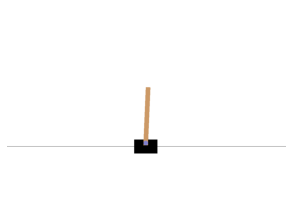Let's see how to interact with an environment. Your agent will need to select an action from an "action space" (the set of possible actions). Let's see what this environment's action space looks like: In : env.action_space  Out: Discrete(2) Yep, just two possible actions: accelerate towards the left or towards the right. Since the pole is leaning toward the right (obs > 0), let's accelerate the cart toward the right: In : action = 1 # accelerate right obs, reward, done, info = env.step(action) obs  Out: array([-0.01261699, 0.19292789, 0.04204097, -0.28092127]) Notice that the cart is now moving toward the right (obs > 0). The pole is still tilted toward the right (obs > 0), but its angular velocity is now negative (obs < 0), so it will likely be tilted toward the left after the next step. In : plot_environment(env) save_fig("cart_pole_plot")  Saving figure cart_pole_plot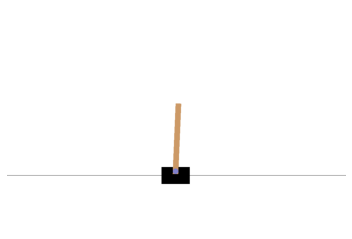Looks like it's doing what we're telling it to do! The environment also tells the agent how much reward it got during the last step: In : reward  Out: 1.0 When the game is over, the environment returns done=True: In : done  Out: False Finally, info is an environment-specific dictionary that can provide some extra information that you may find useful for debugging or for training. For example, in some games it may indicate how many lives the agent has. In : info  Out: {} The sequence of steps between the moment the environment is reset until it is done is called an "episode". At the end of an episode (i.e., when step() returns done=True), you should reset the environment before you continue to use it. In : if done: obs = env.reset()  Now how can we make the poll remain upright? We will need to define a policy for that. This is the strategy that the agent will use to select an action at each step. It can use all the past actions and observations to decide what to do. # A simple hard-coded policy¶ Let's hard code a simple strategy: if the pole is tilting to the left, then push the cart to the left, and vice versa. Let's see if that works: In : env.seed(42) def basic_policy(obs): angle = obs return 0 if angle < 0 else 1 totals = [] for episode in range(500): episode_rewards = 0 obs = env.reset() for step in range(200): action = basic_policy(obs) obs, reward, done, info = env.step(action) episode_rewards += reward if done: break totals.append(episode_rewards)  In : np.mean(totals), np.std(totals), np.min(totals), np.max(totals)  Out: (41.718, 8.858356280936096, 24.0, 68.0) Well, as expected, this strategy is a bit too basic: the best it did was to keep the poll up for only 68 steps. This environment is considered solved when the agent keeps the poll up for 200 steps. Let's visualize one episode: In : env.seed(42) frames = [] obs = env.reset() for step in range(200): img = env.render(mode="rgb_array") frames.append(img) action = basic_policy(obs) obs, reward, done, info = env.step(action) if done: break  Now show the animation: In : def update_scene(num, frames, patch): patch.set_data(frames[num]) return patch, def plot_animation(frames, repeat=False, interval=40): fig = plt.figure() patch = plt.imshow(frames) plt.axis('off') anim = animation.FuncAnimation( fig, update_scene, fargs=(frames, patch), frames=len(frames), repeat=repeat, interval=interval) plt.close() return anim  In : plot_animation(frames)  Clearly the system is unstable and after just a few wobbles, the pole ends up too tilted: game over. We will need to be smarter than that! # Neural Network Policies¶ Let's create a neural network that will take observations as inputs, and output the probabilities of actions to take for each observation. To choose an action, the network will estimate a probability for each action, then we will select an action randomly according to the estimated probabilities. In the case of the Cart-Pole environment, there are just two possible actions (left or right), so we only need one output neuron: it will output the probability p of the action 0 (left), and of course the probability of action 1 (right) will be 1 - p. In : keras.backend.clear_session() tf.random.set_seed(42) np.random.seed(42) n_inputs = 4 # == env.observation_space.shape model = keras.models.Sequential([ keras.layers.Dense(5, activation="elu", input_shape=[n_inputs]), keras.layers.Dense(1, activation="sigmoid"), ])  In this particular environment, the past actions and observations can safely be ignored, since each observation contains the environment's full state. If there were some hidden state then you may need to consider past actions and observations in order to try to infer the hidden state of the environment. For example, if the environment only revealed the position of the cart but not its velocity, you would have to consider not only the current observation but also the previous observation in order to estimate the current velocity. Another example is if the observations are noisy: you may want to use the past few observations to estimate the most likely current state. Our problem is thus as simple as can be: the current observation is noise-free and contains the environment's full state. You may wonder why we plan to pick a random action based on the probability given by the policy network, rather than just picking the action with the highest probability. This approach lets the agent find the right balance between exploring new actions and exploiting the actions that are known to work well. Here's an analogy: suppose you go to a restaurant for the first time, and all the dishes look equally appealing so you randomly pick one. If it turns out to be good, you can increase the probability to order it next time, but you shouldn't increase that probability to 100%, or else you will never try out the other dishes, some of which may be even better than the one you tried. Let's write a small function that will run the model to play one episode, and return the frames so we can display an animation: In : def render_policy_net(model, n_max_steps=200, seed=42): frames = [] env = gym.make("CartPole-v1") env.seed(seed) np.random.seed(seed) obs = env.reset() for step in range(n_max_steps): frames.append(env.render(mode="rgb_array")) left_proba = model.predict(obs.reshape(1, -1)) action = int(np.random.rand() > left_proba) obs, reward, done, info = env.step(action) if done: break env.close() return frames  Now let's look at how well this randomly initialized policy network performs: In : frames = render_policy_net(model) plot_animation(frames)  Yeah... pretty bad. The neural network will have to learn to do better. First let's see if it is capable of learning the basic policy we used earlier: go left if the pole is tilting left, and go right if it is tilting right. We can make the same net play in 50 different environments in parallel (this will give us a diverse training batch at each step), and train for 5000 iterations. We also reset environments when they are done. We train the model using a custom training loop so we can easily use the predictions at each training step to advance the environments. In : n_environments = 50 n_iterations = 5000 envs = [gym.make("CartPole-v1") for _ in range(n_environments)] for index, env in enumerate(envs): env.seed(index) np.random.seed(42) observations = [env.reset() for env in envs] optimizer = keras.optimizers.RMSprop() loss_fn = keras.losses.binary_crossentropy for iteration in range(n_iterations): # if angle < 0, we want proba(left) = 1., or else proba(left) = 0. target_probas = np.array([([1.] if obs < 0 else [0.]) for obs in observations]) with tf.GradientTape() as tape: left_probas = model(np.array(observations)) loss = tf.reduce_mean(loss_fn(target_probas, left_probas)) print("\rIteration: {}, Loss: {:.3f}".format(iteration, loss.numpy()), end="") grads = tape.gradient(loss, model.trainable_variables) optimizer.apply_gradients(zip(grads, model.trainable_variables)) actions = (np.random.rand(n_environments, 1) > left_probas.numpy()).astype(np.int32) for env_index, env in enumerate(envs): obs, reward, done, info = env.step(actions[env_index]) observations[env_index] = obs if not done else env.reset() for env in envs: env.close()  Iteration: 4999, Loss: 0.094 In : frames = render_policy_net(model) plot_animation(frames)  Looks like it learned the policy correctly. Now let's see if it can learn a better policy on its own. One that does not wobble as much. # Policy Gradients¶ To train this neural network we will need to define the target probabilities y. If an action is good we should increase its probability, and conversely if it is bad we should reduce it. But how do we know whether an action is good or bad? The problem is that most actions have delayed effects, so when you win or lose points in an episode, it is not clear which actions contributed to this result: was it just the last action? Or the last 10? Or just one action 50 steps earlier? This is called the credit assignment problem. The Policy Gradients algorithm tackles this problem by first playing multiple episodes, then making the actions in good episodes slightly more likely, while actions in bad episodes are made slightly less likely. First we play, then we go back and think about what we did. Let's start by creating a function to play a single step using the model. We will also pretend for now that whatever action it takes is the right one, so we can compute the loss and its gradients (we will just save these gradients for now, and modify them later depending on how good or bad the action turned out to be): In : def play_one_step(env, obs, model, loss_fn): with tf.GradientTape() as tape: left_proba = model(obs[np.newaxis]) action = (tf.random.uniform([1, 1]) > left_proba) y_target = tf.constant([[1.]]) - tf.cast(action, tf.float32) loss = tf.reduce_mean(loss_fn(y_target, left_proba)) grads = tape.gradient(loss, model.trainable_variables) obs, reward, done, info = env.step(int(action[0, 0].numpy())) return obs, reward, done, grads  If left_proba is high, then action will most likely be False (since a random number uniformally sampled between 0 and 1 will probably not be greater than left_proba). And False means 0 when you cast it to a number, so y_target would be equal to 1 - 0 = 1. In other words, we set the target to 1, meaning we pretend that the probability of going left should have been 100% (so we took the right action). Now let's create another function that will rely on the play_one_step() function to play multiple episodes, returning all the rewards and gradients, for each episode and each step: In : def play_multiple_episodes(env, n_episodes, n_max_steps, model, loss_fn): all_rewards = [] all_grads = [] for episode in range(n_episodes): current_rewards = [] current_grads = [] obs = env.reset() for step in range(n_max_steps): obs, reward, done, grads = play_one_step(env, obs, model, loss_fn) current_rewards.append(reward) current_grads.append(grads) if done: break all_rewards.append(current_rewards) all_grads.append(current_grads) return all_rewards, all_grads  The Policy Gradients algorithm uses the model to play the episode several times (e.g., 10 times), then it goes back and looks at all the rewards, discounts them and normalizes them. So let's create couple functions for that: the first will compute discounted rewards; the second will normalize the discounted rewards across many episodes. In : def discount_rewards(rewards, discount_rate): discounted = np.array(rewards) for step in range(len(rewards) - 2, -1, -1): discounted[step] += discounted[step + 1] * discount_rate return discounted def discount_and_normalize_rewards(all_rewards, discount_rate): all_discounted_rewards = [discount_rewards(rewards, discount_rate) for rewards in all_rewards] flat_rewards = np.concatenate(all_discounted_rewards) reward_mean = flat_rewards.mean() reward_std = flat_rewards.std() return [(discounted_rewards - reward_mean) / reward_std for discounted_rewards in all_discounted_rewards]  Say there were 3 actions, and after each action there was a reward: first 10, then 0, then -50. If we use a discount factor of 80%, then the 3rd action will get -50 (full credit for the last reward), but the 2nd action will only get -40 (80% credit for the last reward), and the 1st action will get 80% of -40 (-32) plus full credit for the first reward (+10), which leads to a discounted reward of -22: In : discount_rewards([10, 0, -50], discount_rate=0.8)  Out: array([-22, -40, -50]) To normalize all discounted rewards across all episodes, we compute the mean and standard deviation of all the discounted rewards, and we subtract the mean from each discounted reward, and divide by the standard deviation: In : discount_and_normalize_rewards([[10, 0, -50], [10, 20]], discount_rate=0.8)  Out: [array([-0.28435071, -0.86597718, -1.18910299]), array([1.26665318, 1.0727777 ])] In : n_iterations = 150 n_episodes_per_update = 10 n_max_steps = 200 discount_rate = 0.95  In : optimizer = keras.optimizers.Adam(lr=0.01) loss_fn = keras.losses.binary_crossentropy  In : keras.backend.clear_session() np.random.seed(42) tf.random.set_seed(42) model = keras.models.Sequential([ keras.layers.Dense(5, activation="elu", input_shape=), keras.layers.Dense(1, activation="sigmoid"), ])  In : env = gym.make("CartPole-v1") env.seed(42); for iteration in range(n_iterations): all_rewards, all_grads = play_multiple_episodes( env, n_episodes_per_update, n_max_steps, model, loss_fn) total_rewards = sum(map(sum, all_rewards)) # Not shown in the book print("\rIteration: {}, mean rewards: {:.1f}".format( # Not shown iteration, total_rewards / n_episodes_per_update), end="") # Not shown all_final_rewards = discount_and_normalize_rewards(all_rewards, discount_rate) all_mean_grads = [] for var_index in range(len(model.trainable_variables)): mean_grads = tf.reduce_mean( [final_reward * all_grads[episode_index][step][var_index] for episode_index, final_rewards in enumerate(all_final_rewards) for step, final_reward in enumerate(final_rewards)], axis=0) all_mean_grads.append(mean_grads) optimizer.apply_gradients(zip(all_mean_grads, model.trainable_variables)) env.close()  Iteration: 149, mean rewards: 197.6 In : frames = render_policy_net(model) plot_animation(frames)  # Markov Chains¶ In : np.random.seed(42) transition_probabilities = [ # shape=[s, s'] [0.7, 0.2, 0.0, 0.1], # from s0 to s0, s1, s2, s3 [0.0, 0.0, 0.9, 0.1], # from s1 to ... [0.0, 1.0, 0.0, 0.0], # from s2 to ... [0.0, 0.0, 0.0, 1.0]] # from s3 to ... n_max_steps = 50 def print_sequence(): current_state = 0 print("States:", end=" ") for step in range(n_max_steps): print(current_state, end=" ") if current_state == 3: break current_state = np.random.choice(range(4), p=transition_probabilities[current_state]) else: print("...", end="") print() for _ in range(10): print_sequence()  States: 0 0 3 States: 0 1 2 1 2 1 2 1 2 1 3 States: 0 1 2 1 2 1 2 1 2 1 2 1 2 1 2 1 2 1 2 1 2 1 3 States: 0 3 States: 0 1 2 1 2 1 2 1 2 1 2 1 2 1 2 1 3 States: 0 1 3 States: 0 1 2 1 2 1 2 1 2 1 2 1 2 1 2 1 2 1 2 1 2 1 2 1 2 1 2 1 2 1 2 1 2 1 2 1 2 1 2 1 2 1 2 1 2 1 2 1 2 1 ... States: 0 0 3 States: 0 0 0 1 2 1 2 1 3 States: 0 1 2 1 2 1 2 1 2 1 2 1 2 1 2 1 2 1 2 1 2 1 3  # Markov Decision Process¶ Let's define some transition probabilities, rewards and possible actions. For example, in state s0, if action a0 is chosen then with proba 0.7 we will go to state s0 with reward +10, with probability 0.3 we will go to state s1 with no reward, and with never go to state s2 (so the transition probabilities are [0.7, 0.3, 0.0], and the rewards are [+10, 0, 0]): In : transition_probabilities = [ # shape=[s, a, s'] [[0.7, 0.3, 0.0], [1.0, 0.0, 0.0], [0.8, 0.2, 0.0]], [[0.0, 1.0, 0.0], None, [0.0, 0.0, 1.0]], [None, [0.8, 0.1, 0.1], None]] rewards = [ # shape=[s, a, s'] [[+10, 0, 0], [0, 0, 0], [0, 0, 0]], [[0, 0, 0], [0, 0, 0], [0, 0, -50]], [[0, 0, 0], [+40, 0, 0], [0, 0, 0]]] possible_actions = [[0, 1, 2], [0, 2], ]  # Q-Value Iteration¶ In : Q_values = np.full((3, 3), -np.inf) # -np.inf for impossible actions for state, actions in enumerate(possible_actions): Q_values[state, actions] = 0.0 # for all possible actions  In : gamma = 0.90 # the discount factor history1 = [] # Not shown in the book (for the figure below) for iteration in range(50): Q_prev = Q_values.copy() history1.append(Q_prev) # Not shown for s in range(3): for a in possible_actions[s]: Q_values[s, a] = np.sum([ transition_probabilities[s][a][sp] * (rewards[s][a][sp] + gamma * np.max(Q_prev[sp])) for sp in range(3)]) history1 = np.array(history1) # Not shown  In : Q_values  Out: array([[18.91891892, 17.02702702, 13.62162162], [ 0. , -inf, -4.87971488], [ -inf, 50.13365013, -inf]]) In : np.argmax(Q_values, axis=1)  Out: array([0, 0, 1]) The optimal policy for this MDP, when using a discount factor of 0.90, is to choose action a0 when in state s0, and choose action a0 when in state s1, and finally choose action a1 (the only possible action) when in state s2. Let's try again with a discount factor of 0.95: In : Q_values = np.full((3, 3), -np.inf) # -np.inf for impossible actions for state, actions in enumerate(possible_actions): Q_values[state, actions] = 0.0 # for all possible actions  In : gamma = 0.95 # the discount factor for iteration in range(50): Q_prev = Q_values.copy() for s in range(3): for a in possible_actions[s]: Q_values[s, a] = np.sum([ transition_probabilities[s][a][sp] * (rewards[s][a][sp] + gamma * np.max(Q_prev[sp])) for sp in range(3)])  In : Q_values  Out: array([[21.73304188, 20.63807938, 16.70138772], [ 0.95462106, -inf, 1.01361207], [ -inf, 53.70728682, -inf]]) In : np.argmax(Q_values, axis=1)  Out: array([0, 2, 1]) Now the policy has changed! In state s1, we now prefer to go through the fire (choose action a2). This is because the discount factor is larger so the agent values the future more, and it is therefore ready to pay an immediate penalty in order to get more future rewards. # Q-Learning¶ Q-Learning works by watching an agent play (e.g., randomly) and gradually improving its estimates of the Q-Values. Once it has accurate Q-Value estimates (or close enough), then the optimal policy consists in choosing the action that has the highest Q-Value (i.e., the greedy policy). We will need to simulate an agent moving around in the environment, so let's define a function to perform some action and get the new state and a reward: In : def step(state, action): probas = transition_probabilities[state][action] next_state = np.random.choice([0, 1, 2], p=probas) reward = rewards[state][action][next_state] return next_state, reward  We also need an exploration policy, which can be any policy, as long as it visits every possible state many times. We will just use a random policy, since the state space is very small: In : def exploration_policy(state): return np.random.choice(possible_actions[state])  Now let's initialize the Q-Values like earlier, and run the Q-Learning algorithm: In : np.random.seed(42) Q_values = np.full((3, 3), -np.inf) for state, actions in enumerate(possible_actions): Q_values[state][actions] = 0 alpha0 = 0.05 # initial learning rate decay = 0.005 # learning rate decay gamma = 0.90 # discount factor state = 0 # initial state history2 = [] # Not shown in the book for iteration in range(10000): history2.append(Q_values.copy()) # Not shown action = exploration_policy(state) next_state, reward = step(state, action) next_value = np.max(Q_values[next_state]) # greedy policy at the next step alpha = alpha0 / (1 + iteration * decay) Q_values[state, action] *= 1 - alpha Q_values[state, action] += alpha * (reward + gamma * next_value) state = next_state history2 = np.array(history2) # Not shown  In : Q_values  Out: array([[18.77621289, 17.2238872 , 13.74543343], [ 0. , -inf, -8.00485647], [ -inf, 49.40208921, -inf]]) In : np.argmax(Q_values, axis=1) # optimal action for each state  Out: array([0, 0, 1]) In : true_Q_value = history1[-1, 0, 0] fig, axes = plt.subplots(1, 2, figsize=(10, 4), sharey=True) axes.set_ylabel("Q-Value$(s_0, a_0)\$", fontsize=14)
axes.set_title("Q-Value Iteration", fontsize=14)
axes.set_title("Q-Learning", fontsize=14)
for ax, width, history in zip(axes, (50, 10000), (history1, history2)):
ax.plot([0, width], [true_Q_value, true_Q_value], "k--")
ax.plot(np.arange(width), history[:, 0, 0], "b-", linewidth=2)
ax.set_xlabel("Iterations", fontsize=14)
ax.axis([0, width, 0, 24])

save_fig("q_value_plot")

Saving figure q_value_plot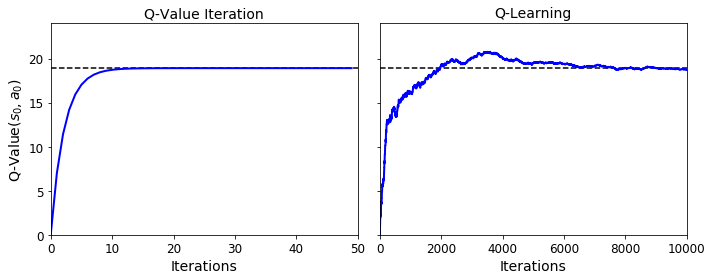# Deep Q-Network¶

Let's build the DQN. Given a state, it will estimate, for each possible action, the sum of discounted future rewards it can expect after it plays that action (but before it sees its outcome):

In :
keras.backend.clear_session()
tf.random.set_seed(42)
np.random.seed(42)

env = gym.make("CartPole-v1")
input_shape =  # == env.observation_space.shape
n_outputs = 2 # == env.action_space.n

model = keras.models.Sequential([
keras.layers.Dense(32, activation="elu", input_shape=input_shape),
keras.layers.Dense(32, activation="elu"),
keras.layers.Dense(n_outputs)
])


To select an action using this DQN, we just pick the action with the largest predicted Q-value. However, to ensure that the agent explores the environment, we choose a random action with probability epsilon.

In :
def epsilon_greedy_policy(state, epsilon=0):
if np.random.rand() < epsilon:
return np.random.randint(n_outputs)
else:
Q_values = model.predict(state[np.newaxis])
return np.argmax(Q_values)


We will also need a replay memory. It will contain the agent's experiences, in the form of tuples: (obs, action, reward, next_obs, done). We can use the deque class for that (but make sure to check out DeepMind's excellent Reverb library for a much more robust implementation of experience replay):

In :
from collections import deque

replay_memory = deque(maxlen=2000)


And let's create a function to sample experiences from the replay memory. It will return 5 NumPy arrays: [obs, actions, rewards, next_obs, dones].

In :
def sample_experiences(batch_size):
indices = np.random.randint(len(replay_memory), size=batch_size)
batch = [replay_memory[index] for index in indices]
states, actions, rewards, next_states, dones = [
np.array([experience[field_index] for experience in batch])
for field_index in range(5)]
return states, actions, rewards, next_states, dones


Now we can create a function that will use the DQN to play one step, and record its experience in the replay memory:

In :
def play_one_step(env, state, epsilon):
action = epsilon_greedy_policy(state, epsilon)
next_state, reward, done, info = env.step(action)
replay_memory.append((state, action, reward, next_state, done))
return next_state, reward, done, info


Lastly, let's create a function that will sample some experiences from the replay memory and perform a training step:

Note: the first 3 releases of the 2nd edition were missing the reshape() operation which converts target_Q_values to a column vector (this is required by the loss_fn()).

In :
batch_size = 32
discount_rate = 0.95
optimizer = keras.optimizers.Adam(lr=1e-3)
loss_fn = keras.losses.mean_squared_error

def training_step(batch_size):
experiences = sample_experiences(batch_size)
states, actions, rewards, next_states, dones = experiences
next_Q_values = model.predict(next_states)
max_next_Q_values = np.max(next_Q_values, axis=1)
target_Q_values = (rewards +
(1 - dones) * discount_rate * max_next_Q_values)
target_Q_values = target_Q_values.reshape(-1, 1)
mask = tf.one_hot(actions, n_outputs)
with tf.GradientTape() as tape:
all_Q_values = model(states)
Q_values = tf.reduce_sum(all_Q_values * mask, axis=1, keepdims=True)
loss = tf.reduce_mean(loss_fn(target_Q_values, Q_values))
grads = tape.gradient(loss, model.trainable_variables)
optimizer.apply_gradients(zip(grads, model.trainable_variables))


And now, let's train the model!

In :
env.seed(42)
np.random.seed(42)
tf.random.set_seed(42)

rewards = []
best_score = 0

In :
for episode in range(600):
obs = env.reset()
for step in range(200):
epsilon = max(1 - episode / 500, 0.01)
obs, reward, done, info = play_one_step(env, obs, epsilon)
if done:
break
rewards.append(step) # Not shown in the book
if step > best_score: # Not shown
best_weights = model.get_weights() # Not shown
best_score = step # Not shown
print("\rEpisode: {}, Steps: {}, eps: {:.3f}".format(episode, step + 1, epsilon), end="") # Not shown
if episode > 50:
training_step(batch_size)

model.set_weights(best_weights)

Episode: 599, Steps: 19, eps: 0.0108
In :
plt.figure(figsize=(8, 4))
plt.plot(rewards)
plt.xlabel("Episode", fontsize=14)
plt.ylabel("Sum of rewards", fontsize=14)
save_fig("dqn_rewards_plot")
plt.show()

Saving figure dqn_rewards_plot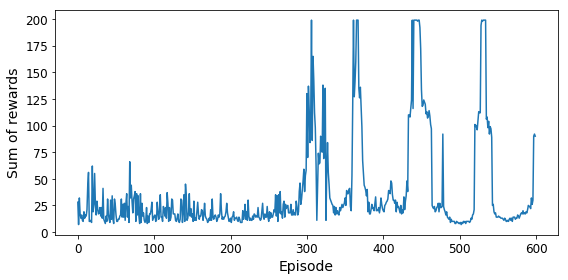In :
env.seed(42)
state = env.reset()

frames = []

for step in range(200):
action = epsilon_greedy_policy(state)
state, reward, done, info = env.step(action)
if done:
break
img = env.render(mode="rgb_array")
frames.append(img)

plot_animation(frames)


Not bad at all!

## Double DQN¶

In :
keras.backend.clear_session()
tf.random.set_seed(42)
np.random.seed(42)

model = keras.models.Sequential([
keras.layers.Dense(32, activation="elu", input_shape=),
keras.layers.Dense(32, activation="elu"),
keras.layers.Dense(n_outputs)
])

target = keras.models.clone_model(model)
target.set_weights(model.get_weights())

In :
batch_size = 32
discount_rate = 0.95
optimizer = keras.optimizers.Adam(lr=1e-3)
loss_fn = keras.losses.Huber()

def training_step(batch_size):
experiences = sample_experiences(batch_size)
states, actions, rewards, next_states, dones = experiences
next_Q_values = model.predict(next_states)
best_next_actions = np.argmax(next_Q_values, axis=1)
next_mask = tf.one_hot(best_next_actions, n_outputs).numpy()
next_best_Q_values = (target.predict(next_states) * next_mask).sum(axis=1)
target_Q_values = (rewards +
(1 - dones) * discount_rate * next_best_Q_values)
target_Q_values = target_Q_values.reshape(-1, 1)
mask = tf.one_hot(actions, n_outputs)
with tf.GradientTape() as tape:
all_Q_values = model(states)
Q_values = tf.reduce_sum(all_Q_values * mask, axis=1, keepdims=True)
loss = tf.reduce_mean(loss_fn(target_Q_values, Q_values))
grads = tape.gradient(loss, model.trainable_variables)
optimizer.apply_gradients(zip(grads, model.trainable_variables))

In :
replay_memory = deque(maxlen=2000)

In :
env.seed(42)
np.random.seed(42)
tf.random.set_seed(42)

rewards = []
best_score = 0

for episode in range(600):
obs = env.reset()
for step in range(200):
epsilon = max(1 - episode / 500, 0.01)
obs, reward, done, info = play_one_step(env, obs, epsilon)
if done:
break
rewards.append(step)
if step > best_score:
best_weights = model.get_weights()
best_score = step
print("\rEpisode: {}, Steps: {}, eps: {:.3f}".format(episode, step + 1, epsilon), end="")
if episode > 50:
training_step(batch_size)
if episode % 50 == 0:
target.set_weights(model.get_weights())
# Alternatively, you can do soft updates at each step:
#if episode > 50:
#target_weights = target.get_weights()
#online_weights = model.get_weights()
#for index in range(len(target_weights)):
#    target_weights[index] = 0.99 * target_weights[index] + 0.01 * online_weights[index]
#target.set_weights(target_weights)

model.set_weights(best_weights)

Episode: 599, Steps: 49, eps: 0.0100
In :
plt.figure(figsize=(8, 4))
plt.plot(rewards)
plt.xlabel("Episode", fontsize=14)
plt.ylabel("Sum of rewards", fontsize=14)
save_fig("double_dqn_rewards_plot")
plt.show()

Saving figure dqn_rewards_plot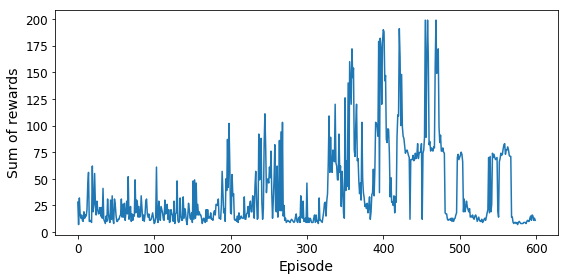In :
env.seed(42)
state = env.reset()

frames = []

for step in range(200):
action = epsilon_greedy_policy(state)
state, reward, done, info = env.step(action)
if done:
break
img = env.render(mode="rgb_array")
frames.append(img)

plot_animation(frames)


# Dueling Double DQN¶

In :
keras.backend.clear_session()
tf.random.set_seed(42)
np.random.seed(42)

K = keras.backend
input_states = keras.layers.Input(shape=)
hidden1 = keras.layers.Dense(32, activation="elu")(input_states)
hidden2 = keras.layers.Dense(32, activation="elu")(hidden1)
state_values = keras.layers.Dense(1)(hidden2)
raw_advantages = keras.layers.Dense(n_outputs)(hidden2)
advantages = raw_advantages - K.max(raw_advantages, axis=1, keepdims=True)
Q_values = state_values + advantages
model = keras.models.Model(inputs=[input_states], outputs=[Q_values])

target = keras.models.clone_model(model)
target.set_weights(model.get_weights())

In :
batch_size = 32
discount_rate = 0.95
optimizer = keras.optimizers.Adam(lr=1e-2)
loss_fn = keras.losses.Huber()

def training_step(batch_size):
experiences = sample_experiences(batch_size)
states, actions, rewards, next_states, dones = experiences
next_Q_values = model.predict(next_states)
best_next_actions = np.argmax(next_Q_values, axis=1)
next_mask = tf.one_hot(best_next_actions, n_outputs).numpy()
next_best_Q_values = (target.predict(next_states) * next_mask).sum(axis=1)
target_Q_values = (rewards +
(1 - dones) * discount_rate * next_best_Q_values)
target_Q_values = target_Q_values.reshape(-1, 1)
mask = tf.one_hot(actions, n_outputs)
with tf.GradientTape() as tape:
all_Q_values = model(states)
Q_values = tf.reduce_sum(all_Q_values * mask, axis=1, keepdims=True)
loss = tf.reduce_mean(loss_fn(target_Q_values, Q_values))
grads = tape.gradient(loss, model.trainable_variables)
optimizer.apply_gradients(zip(grads, model.trainable_variables))

In :
replay_memory = deque(maxlen=2000)

In :
env.seed(42)
np.random.seed(42)
tf.random.set_seed(42)

rewards = []
best_score = 0

for episode in range(600):
obs = env.reset()
for step in range(200):
epsilon = max(1 - episode / 500, 0.01)
obs, reward, done, info = play_one_step(env, obs, epsilon)
if done:
break
rewards.append(step)
if step > best_score:
best_weights = model.get_weights()
best_score = step
print("\rEpisode: {}, Steps: {}, eps: {:.3f}".format(episode, step + 1, epsilon), end="")
if episode > 50:
training_step(batch_size)
if episode % 200 == 0:
target.set_weights(model.get_weights())

model.set_weights(best_weights)

Episode: 599, Steps: 74, eps: 0.0100
In :
plt.plot(rewards)
plt.xlabel("Episode")
plt.ylabel("Sum of rewards")
plt.show()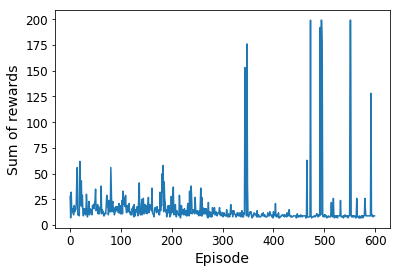In :
env.seed(42)
state = env.reset()

frames = []

for step in range(200):
action = epsilon_greedy_policy(state)
state, reward, done, info = env.step(action)
if done:
break
img = env.render(mode="rgb_array")
frames.append(img)

plot_animation(frames)


This looks like a pretty robust agent!

In :
env.close()


# Using TF-Agents to Beat Breakout¶

Let's use TF-Agents to create an agent that will learn to play Breakout. We will use the Deep Q-Learning algorithm, so you can easily compare the components with the previous implementation, but TF-Agents implements many other (and more sophisticated) algorithms!

## TF-Agents Environments¶

In :
tf.random.set_seed(42)
np.random.seed(42)

In :
from tf_agents.environments import suite_gym

env = suite_gym.load("Breakout-v4")
env

Out:
<tf_agents.environments.wrappers.TimeLimit at 0x7ff79b28f590>
In :
env.gym

Out:
<gym.envs.atari.atari_env.AtariEnv at 0x7ff889a2fe90>
In :
env.seed(42)
env.reset()

Out:
TimeStep(step_type=array(0, dtype=int32), reward=array(0., dtype=float32), discount=array(1., dtype=float32), observation=array([[[0, 0, 0],
[0, 0, 0],
[0, 0, 0],
...,
[0, 0, 0],
[0, 0, 0],
[0, 0, 0]],

[[0, 0, 0],
[0, 0, 0],
[0, 0, 0],
...,
[0, 0, 0],
[0, 0, 0],
[0, 0, 0]],

[[0, 0, 0],
[0, 0, 0],
[0, 0, 0],
...,
[0, 0, 0],
[0, 0, 0],
[0, 0, 0]],

...,

[[0, 0, 0],
[0, 0, 0],
[0, 0, 0],
...,
[0, 0, 0],
[0, 0, 0],
[0, 0, 0]],

[[0, 0, 0],
[0, 0, 0],
[0, 0, 0],
...,
[0, 0, 0],
[0, 0, 0],
[0, 0, 0]],

[[0, 0, 0],
[0, 0, 0],
[0, 0, 0],
...,
[0, 0, 0],
[0, 0, 0],
[0, 0, 0]]], dtype=uint8))
In :
env.step(1) # Fire

Out:
TimeStep(step_type=array(1, dtype=int32), reward=array(0., dtype=float32), discount=array(1., dtype=float32), observation=array([[[0, 0, 0],
[0, 0, 0],
[0, 0, 0],
...,
[0, 0, 0],
[0, 0, 0],
[0, 0, 0]],

[[0, 0, 0],
[0, 0, 0],
[0, 0, 0],
...,
[0, 0, 0],
[0, 0, 0],
[0, 0, 0]],

[[0, 0, 0],
[0, 0, 0],
[0, 0, 0],
...,
[0, 0, 0],
[0, 0, 0],
[0, 0, 0]],

...,

[[0, 0, 0],
[0, 0, 0],
[0, 0, 0],
...,
[0, 0, 0],
[0, 0, 0],
[0, 0, 0]],

[[0, 0, 0],
[0, 0, 0],
[0, 0, 0],
...,
[0, 0, 0],
[0, 0, 0],
[0, 0, 0]],

[[0, 0, 0],
[0, 0, 0],
[0, 0, 0],
...,
[0, 0, 0],
[0, 0, 0],
[0, 0, 0]]], dtype=uint8))
In :
img = env.render(mode="rgb_array")

plt.figure(figsize=(6, 8))
plt.imshow(img)
plt.axis("off")
save_fig("breakout_plot")
plt.show()

Saving figure breakout_plot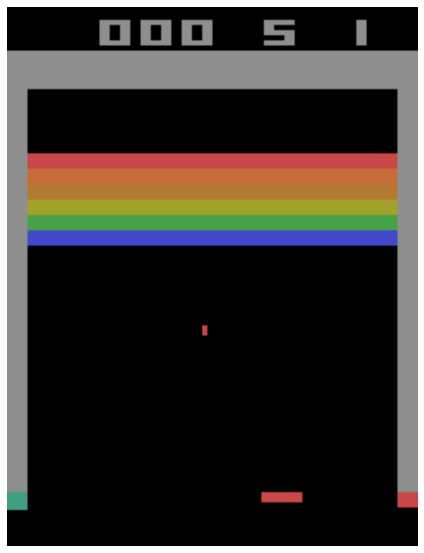In :
env.current_time_step()

Out:
TimeStep(step_type=array(1, dtype=int32), reward=array(0., dtype=float32), discount=array(1., dtype=float32), observation=array([[[0, 0, 0],
[0, 0, 0],
[0, 0, 0],
...,
[0, 0, 0],
[0, 0, 0],
[0, 0, 0]],

[[0, 0, 0],
[0, 0, 0],
[0, 0, 0],
...,
[0, 0, 0],
[0, 0, 0],
[0, 0, 0]],

[[0, 0, 0],
[0, 0, 0],
[0, 0, 0],
...,
[0, 0, 0],
[0, 0, 0],
[0, 0, 0]],

...,

[[0, 0, 0],
[0, 0, 0],
[0, 0, 0],
...,
[0, 0, 0],
[0, 0, 0],
[0, 0, 0]],

[[0, 0, 0],
[0, 0, 0],
[0, 0, 0],
...,
[0, 0, 0],
[0, 0, 0],
[0, 0, 0]],

[[0, 0, 0],
[0, 0, 0],
[0, 0, 0],
...,
[0, 0, 0],
[0, 0, 0],
[0, 0, 0]]], dtype=uint8))

## Environment Specifications¶

In :
env.observation_spec()

Out:
BoundedArraySpec(shape=(210, 160, 3), dtype=dtype('uint8'), name='observation', minimum=0, maximum=255)
In :
env.action_spec()

Out:
BoundedArraySpec(shape=(), dtype=dtype('int64'), name='action', minimum=0, maximum=3)
In :
env.time_step_spec()

Out:
TimeStep(step_type=ArraySpec(shape=(), dtype=dtype('int32'), name='step_type'), reward=ArraySpec(shape=(), dtype=dtype('float32'), name='reward'), discount=BoundedArraySpec(shape=(), dtype=dtype('float32'), name='discount', minimum=0.0, maximum=1.0), observation=BoundedArraySpec(shape=(210, 160, 3), dtype=dtype('uint8'), name='observation', minimum=0, maximum=255))

## Environment Wrappers¶

You can wrap a TF-Agents environments in a TF-Agents wrapper:

In :
from tf_agents.environments.wrappers import ActionRepeat

repeating_env = ActionRepeat(env, times=4)
repeating_env

Out:
<tf_agents.environments.wrappers.ActionRepeat at 0x7ff889aa4f50>
In :
repeating_env.unwrapped

Out:
<gym.envs.atari.atari_env.AtariEnv at 0x7ff889a2fe90>

Here is the list of available wrappers:

In :
import tf_agents.environments.wrappers

for name in dir(tf_agents.environments.wrappers):
obj = getattr(tf_agents.environments.wrappers, name)
if hasattr(obj, "__base__") and issubclass(obj, tf_agents.environments.wrappers.PyEnvironmentBaseWrapper):
print("{:27s} {}".format(name, obj.__doc__.split("\n")))

ActionClipWrapper           Wraps an environment and clips actions to spec before applying.
ActionDiscretizeWrapper     Wraps an environment with continuous actions and discretizes them.
ActionOffsetWrapper         Offsets actions to be zero-based.
ActionRepeat                Repeates actions over n-steps while acummulating the received reward.
FlattenObservationsWrapper  Wraps an environment and flattens nested multi-dimensional observations.
GoalReplayEnvWrapper        Adds a goal to the observation, used for HER (Hindsight Experience Replay).
HistoryWrapper              Adds observation and action history to the environment's observations.
ObservationFilterWrapper    Filters observations based on an array of indexes.
OneHotActionWrapper         Converts discrete action to one_hot format.
PerformanceProfiler         End episodes after specified number of steps.
PyEnvironmentBaseWrapper    PyEnvironment wrapper forwards calls to the given environment.
RunStats                    Wrapper that accumulates run statistics as the environment iterates.
TimeLimit                   End episodes after specified number of steps.


The suite_gym.load() function can create an env and wrap it for you, both with TF-Agents environment wrappers and Gym environment wrappers (the latter are applied first).

In :
from functools import partial
from gym.wrappers import TimeLimit

limited_repeating_env = suite_gym.load(
"Breakout-v4",
gym_env_wrappers=[partial(TimeLimit, max_episode_steps=10000)],
env_wrappers=[partial(ActionRepeat, times=4)],
)

In :
limited_repeating_env

Out:
<tf_agents.environments.wrappers.ActionRepeat at 0x7ff89feac510>

Create an Atari Breakout environment, and wrap it to apply the default Atari preprocessing steps:

In :
limited_repeating_env.unwrapped

Out:
<gym.envs.atari.atari_env.AtariEnv at 0x7ff8cfe4dd90>
In :
from tf_agents.environments import suite_atari
from tf_agents.environments.atari_preprocessing import AtariPreprocessing
from tf_agents.environments.atari_wrappers import FrameStack4

max_episode_steps = 27000 # <=> 108k ALE frames since 1 step = 4 frames
environment_name = "BreakoutNoFrameskip-v4"

env = suite_atari.load(
environment_name,
max_episode_steps=max_episode_steps,
gym_env_wrappers=[AtariPreprocessing, FrameStack4])

In :
env

Out:
<tf_agents.environments.atari_wrappers.AtariTimeLimit at 0x7ff8bd493650>

Play a few steps just to see what happens:

In :
env.seed(42)
env.reset()
time_step = env.step(1) # FIRE
for _ in range(4):
time_step = env.step(3) # LEFT

In :
def plot_observation(obs):
# Since there are only 3 color channels, you cannot display 4 frames
# with one primary color per frame. So this code computes the delta between
# the current frame and the mean of the other frames, and it adds this delta
# to the red and blue channels to get a pink color for the current frame.
obs = obs.astype(np.float32)
img = obs[..., :3]
current_frame_delta = np.maximum(obs[..., 3] - obs[..., :3].mean(axis=-1), 0.)
img[..., 0] += current_frame_delta
img[..., 2] += current_frame_delta
img = np.clip(img / 150, 0, 1)
plt.imshow(img)
plt.axis("off")

In :
plt.figure(figsize=(6, 6))
plot_observation(time_step.observation)
save_fig("preprocessed_breakout_plot")
plt.show()

Saving figure preprocessed_breakout_plot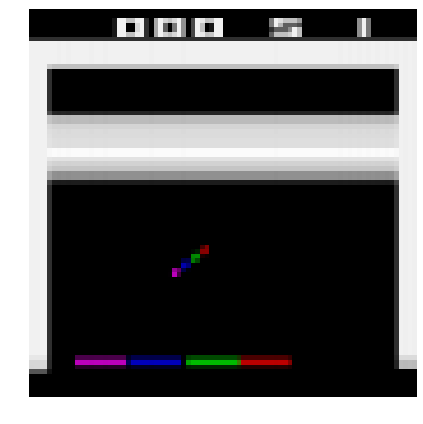Convert the Python environment to a TF environment:

In :
from tf_agents.environments.tf_py_environment import TFPyEnvironment

tf_env = TFPyEnvironment(env)


## Creating the DQN¶

Create a small class to normalize the observations. Images are stored using bytes from 0 to 255 to use less RAM, but we want to pass floats from 0.0 to 1.0 to the neural network:

Create the Q-Network:

In :
from tf_agents.networks.q_network import QNetwork

preprocessing_layer = keras.layers.Lambda(
lambda obs: tf.cast(obs, np.float32) / 255.)
conv_layer_params=[(32, (8, 8), 4), (64, (4, 4), 2), (64, (3, 3), 1)]
fc_layer_params=

q_net = QNetwork(
tf_env.observation_spec(),
tf_env.action_spec(),
preprocessing_layers=preprocessing_layer,
conv_layer_params=conv_layer_params,
fc_layer_params=fc_layer_params)


Create the DQN Agent:

In :
from tf_agents.agents.dqn.dqn_agent import DqnAgent

train_step = tf.Variable(0)
update_period = 4 # run a training step every 4 collect steps
optimizer = keras.optimizers.RMSprop(lr=2.5e-4, rho=0.95, momentum=0.0,
epsilon=0.00001, centered=True)
epsilon_fn = keras.optimizers.schedules.PolynomialDecay(
initial_learning_rate=1.0, # initial ε
decay_steps=250000 // update_period, # <=> 1,000,000 ALE frames
end_learning_rate=0.01) # final ε
agent = DqnAgent(tf_env.time_step_spec(),
tf_env.action_spec(),
q_network=q_net,
optimizer=optimizer,
target_update_period=2000, # <=> 32,000 ALE frames
td_errors_loss_fn=keras.losses.Huber(reduction="none"),
gamma=0.99, # discount factor
train_step_counter=train_step,
epsilon_greedy=lambda: epsilon_fn(train_step))
agent.initialize()


Create the replay buffer (this will use a lot of RAM, so please reduce the buffer size if you get an out-of-memory error):

In :
from tf_agents.replay_buffers import tf_uniform_replay_buffer

replay_buffer = tf_uniform_replay_buffer.TFUniformReplayBuffer(
data_spec=agent.collect_data_spec,
batch_size=tf_env.batch_size,
max_length=1000000) # reduce if OOM error

replay_buffer_observer = replay_buffer.add_batch


Create a simple custom observer that counts and displays the number of times it is called (except when it is passed a trajectory that represents the boundary between two episodes, as this does not count as a step):

In :
class ShowProgress:
def __init__(self, total):
self.counter = 0
self.total = total
def __call__(self, trajectory):
if not trajectory.is_boundary():
self.counter += 1
if self.counter % 100 == 0:
print("\r{}/{}".format(self.counter, self.total), end="")


Let's add some training metrics:

In :
from tf_agents.metrics import tf_metrics

train_metrics = [
tf_metrics.NumberOfEpisodes(),
tf_metrics.EnvironmentSteps(),
tf_metrics.AverageReturnMetric(),
tf_metrics.AverageEpisodeLengthMetric(),
]

In :
train_metrics.result()

Out:
<tf.Tensor: shape=(), dtype=int64, numpy=0>
In :
from tf_agents.eval.metric_utils import log_metrics
import logging
logging.getLogger().setLevel(logging.INFO)
log_metrics(train_metrics)

INFO:absl:
NumberOfEpisodes = 0
EnvironmentSteps = 0
AverageReturn = 0.0
AverageEpisodeLength = 0.0


Create the collect driver:

In :
from tf_agents.drivers.dynamic_step_driver import DynamicStepDriver

collect_driver = DynamicStepDriver(
tf_env,
agent.collect_policy,
observers=[replay_buffer_observer] + train_metrics,
num_steps=update_period) # collect 4 steps for each training iteration


Collect the initial experiences, before training:

In :
from tf_agents.policies.random_tf_policy import RandomTFPolicy

initial_collect_policy = RandomTFPolicy(tf_env.time_step_spec(),
tf_env.action_spec())
init_driver = DynamicStepDriver(
tf_env,
initial_collect_policy,
observers=[replay_buffer.add_batch, ShowProgress(20000)],
num_steps=20000) # <=> 80,000 ALE frames
final_time_step, final_policy_state = init_driver.run()

20000/20000

Let's sample 2 sub-episodes, with 3 time steps each and display them:

Note: replay_buffer.get_next() is deprecated. We must use replay_buffer.as_dataset(..., single_deterministic_pass=False) instead.

In :
tf.random.set_seed(93) # chosen to show an example of trajectory at the end of an episode

#trajectories, buffer_info = replay_buffer.get_next(
#    sample_batch_size=2, num_steps=3)

trajectories, buffer_info = next(iter(replay_buffer.as_dataset(
sample_batch_size=2,
num_steps=3,
single_deterministic_pass=False)))

In :
trajectories._fields

Out:
('step_type',
'observation',
'action',
'policy_info',
'next_step_type',
'reward',
'discount')
In :
trajectories.observation.shape

Out:
TensorShape([2, 3, 84, 84, 4])
In :
from tf_agents.trajectories.trajectory import to_transition

time_steps, action_steps, next_time_steps = to_transition(trajectories)
time_steps.observation.shape

Out:
TensorShape([2, 2, 84, 84, 4])
In :
trajectories.step_type.numpy()

Out:
array([[1, 1, 1],
[1, 1, 1]], dtype=int32)
In :
plt.figure(figsize=(10, 6.8))
for row in range(2):
for col in range(3):
plt.subplot(2, 3, row * 3 + col + 1)
plot_observation(trajectories.observation[row, col].numpy())
plt.subplots_adjust(left=0, right=1, bottom=0, top=1, hspace=0, wspace=0.02)
save_fig("sub_episodes_plot")
plt.show()

Saving figure sub_episodes_plot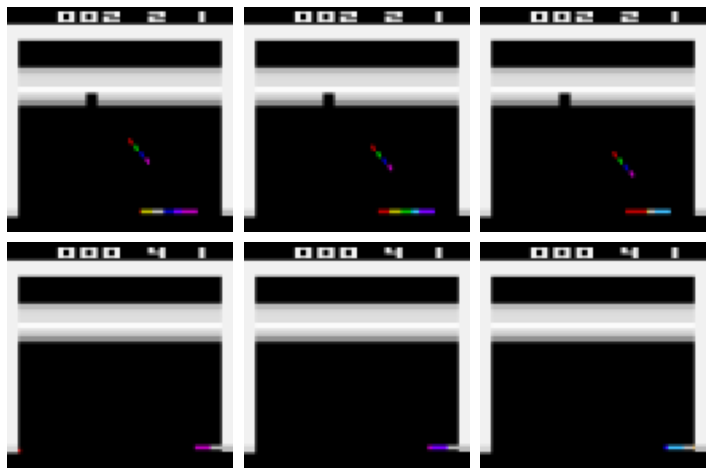Now let's create the dataset:

In :
dataset = replay_buffer.as_dataset(
sample_batch_size=64,
num_steps=2,
num_parallel_calls=3).prefetch(3)


Convert the main functions to TF Functions for better performance:

In :
from tf_agents.utils.common import function

collect_driver.run = function(collect_driver.run)
agent.train = function(agent.train)


And now we are ready to run the main loop!

In :
def train_agent(n_iterations):
time_step = None
policy_state = agent.collect_policy.get_initial_state(tf_env.batch_size)
iterator = iter(dataset)
for iteration in range(n_iterations):
time_step, policy_state = collect_driver.run(time_step, policy_state)
trajectories, buffer_info = next(iterator)
train_loss = agent.train(trajectories)
print("\r{} loss:{:.5f}".format(
iteration, train_loss.loss.numpy()), end="")
if iteration % 1000 == 0:
log_metrics(train_metrics)


Run the next cell to train the agent for 10,000 steps. Then look at its behavior by running the following cell. You can run these two cells as many times as you wish. The agent will keep improving!

In :
train_agent(n_iterations=10000)

INFO:absl:
NumberOfEpisodes = 0
EnvironmentSteps = 4
AverageReturn = 0.0
AverageEpisodeLength = 0.0

998 loss:0.00024
INFO:absl:
NumberOfEpisodes = 24
EnvironmentSteps = 4004
AverageReturn = 0.699999988079071
AverageEpisodeLength = 162.39999389648438

1997 loss:0.00029
INFO:absl:
NumberOfEpisodes = 48
EnvironmentSteps = 8004
AverageReturn = 1.2000000476837158
AverageEpisodeLength = 180.39999389648438

2998 loss:0.00251
INFO:absl:
NumberOfEpisodes = 69
EnvironmentSteps = 12004
AverageReturn = 1.2999999523162842
AverageEpisodeLength = 183.0

3999 loss:0.00029
INFO:absl:
NumberOfEpisodes = 92
EnvironmentSteps = 16004
AverageReturn = 0.800000011920929
AverageEpisodeLength = 167.6999969482422

4996 loss:0.00021
INFO:absl:
NumberOfEpisodes = 114
EnvironmentSteps = 20004
AverageReturn = 1.100000023841858
AverageEpisodeLength = 177.0

5995 loss:0.00009
INFO:absl:
NumberOfEpisodes = 134
EnvironmentSteps = 24004
AverageReturn = 1.399999976158142
AverageEpisodeLength = 198.6999969482422

6999 loss:0.00003
INFO:absl:
NumberOfEpisodes = 156
EnvironmentSteps = 28004
AverageReturn = 1.100000023841858
AverageEpisodeLength = 178.60000610351562

7997 loss:0.00151
INFO:absl:
NumberOfEpisodes = 177
EnvironmentSteps = 32004
AverageReturn = 1.899999976158142
AverageEpisodeLength = 208.60000610351562

8996 loss:0.00002
INFO:absl:
NumberOfEpisodes = 198
EnvironmentSteps = 36004
AverageReturn = 1.7000000476837158
AverageEpisodeLength = 204.89999389648438

9999 loss:0.00006
In :
frames = []
def save_frames(trajectory):
global frames
frames.append(tf_env.pyenv.envs.render(mode="rgb_array"))

prev_lives = tf_env.pyenv.envs.ale.lives()
def reset_and_fire_on_life_lost(trajectory):
global prev_lives
lives = tf_env.pyenv.envs.ale.lives()
if prev_lives != lives:
tf_env.reset()
tf_env.pyenv.envs.step(1)
prev_lives = lives

watch_driver = DynamicStepDriver(
tf_env,
agent.policy,
observers=[save_frames, reset_and_fire_on_life_lost, ShowProgress(1000)],
num_steps=1000)
final_time_step, final_policy_state = watch_driver.run()

plot_animation(frames)


If you want to save an animated GIF to show off your agent to your friends, here's one way to do it:

In :
import PIL

image_path = os.path.join("images", "rl", "breakout.gif")
frame_images = [PIL.Image.fromarray(frame) for frame in frames[:150]]
frame_images.save(image_path, format='GIF',
append_images=frame_images[1:],
save_all=True,
duration=30,
loop=0)

In :
%%html
<img src="images/rl/breakout.gif" /># Extra material¶

## Deque vs Rotating List¶

The deque class offers fast append, but fairly slow random access (for large replay memories):

In :
from collections import deque
np.random.seed(42)

mem = deque(maxlen=1000000)
for i in range(1000000):
mem.append(i)
[mem[i] for i in np.random.randint(1000000, size=5)]

Out:
[121958, 671155, 131932, 365838, 259178]
In :
%timeit mem.append(1)

47.4 ns ± 3.02 ns per loop (mean ± std. dev. of 7 runs, 10000000 loops each)

In :
%timeit [mem[i] for i in np.random.randint(1000000, size=5)]

182 µs ± 6.9 µs per loop (mean ± std. dev. of 7 runs, 1000 loops each)


Alternatively, you could use a rotating list like this ReplayMemory class. This would make random access faster for large replay memories:

In :
class ReplayMemory:
def __init__(self, max_size):
self.buffer = np.empty(max_size, dtype=np.object)
self.max_size = max_size
self.index = 0
self.size = 0

def append(self, obj):
self.buffer[self.index] = obj
self.size = min(self.size + 1, self.max_size)
self.index = (self.index + 1) % self.max_size

def sample(self, batch_size):
indices = np.random.randint(self.size, size=batch_size)
return self.buffer[indices]

In :
mem = ReplayMemory(max_size=1000000)
for i in range(1000000):
mem.append(i)
mem.sample(5)

Out:
array([757386, 904203, 190588, 595754, 865356], dtype=object)
In :
%timeit mem.append(1)

519 ns ± 17.8 ns per loop (mean ± std. dev. of 7 runs, 1000000 loops each)

In :
%timeit mem.sample(5)

9.24 µs ± 227 ns per loop (mean ± std. dev. of 7 runs, 100000 loops each)


## Creating a Custom TF-Agents Environment¶

To create a custom TF-Agent environment, you just need to write a class that inherits from the PyEnvironment class and implements a few methods. For example, the following minimal environment represents a simple 4x4 grid. The agent starts in one corner (0,0) and must move to the opposite corner (3,3). The episode is done if the agent reaches the goal (it gets a +10 reward) or if the agent goes out of bounds (-1 reward). The actions are up (0), down (1), left (2) and right (3).

In :
class MyEnvironment(tf_agents.environments.py_environment.PyEnvironment):
def __init__(self, discount=1.0):
super().__init__()
self._action_spec = tf_agents.specs.BoundedArraySpec(
shape=(), dtype=np.int32, name="action", minimum=0, maximum=3)
self._observation_spec = tf_agents.specs.BoundedArraySpec(
shape=(4, 4), dtype=np.int32, name="observation", minimum=0, maximum=1)
self.discount = discount

def action_spec(self):
return self._action_spec

def observation_spec(self):
return self._observation_spec

def _reset(self):
self._state = np.zeros(2, dtype=np.int32)
obs = np.zeros((4, 4), dtype=np.int32)
obs[self._state, self._state] = 1
return tf_agents.trajectories.time_step.restart(obs)

def _step(self, action):
self._state += [(-1, 0), (+1, 0), (0, -1), (0, +1)][action]
reward = 0
obs = np.zeros((4, 4), dtype=np.int32)
done = (self._state.min() < 0 or self._state.max() > 3)
if not done:
obs[self._state, self._state] = 1
if done or np.all(self._state == np.array([3, 3])):
reward = -1 if done else +10
return tf_agents.trajectories.time_step.termination(obs, reward)
else:
return tf_agents.trajectories.time_step.transition(obs, reward,
self.discount)


The action and observation specs will generally be instances of the ArraySpec or BoundedArraySpec classes from the tf_agents.specs package (check out the other specs in this package as well). Optionally, you can also define a render() method, a close() method to free resources, as well as a time_step_spec() method if you don't want the reward and discount to be 32-bit float scalars. Note that the base class takes care of keeping track of the current time step, which is why we must implement _reset() and _step() rather than reset() and step().

In :
my_env = MyEnvironment()
time_step = my_env.reset()
time_step

Out:
TimeStep(step_type=array(0, dtype=int32), reward=array(0., dtype=float32), discount=array(1., dtype=float32), observation=array([[1, 0, 0, 0],
[0, 0, 0, 0],
[0, 0, 0, 0],
[0, 0, 0, 0]], dtype=int32))
In :
time_step = my_env.step(1)
time_step

Out:
TimeStep(step_type=array(1, dtype=int32), reward=array(0., dtype=float32), discount=array(1., dtype=float32), observation=array([[0, 0, 0, 0],
[1, 0, 0, 0],
[0, 0, 0, 0],
[0, 0, 0, 0]], dtype=int32))
In [ ]: